## Signatures of radioactive isotopes in the $r$-processLA-UR-21-30370

### Matthew Mumpower

Tuesday October 19$^{th}$ 2021Center for Theoretical
ASTROPHYSICSLos Alamos National Laboratory Caveat

The submitted materials have been authored by an employee or employees of Triad National Security, LLC (Triad) under contract with the U.S. Department of Energy/National Nuclear Security Administration (DOE/NNSA).

Accordingly, the U.S. Government retains an irrevocable, nonexclusive, royaltyfree license to publish, translate, reproduce, use, or dispose of the published form of the work and to authorize others to do the same for U.S. Government purposes.

## What is the $r$-process?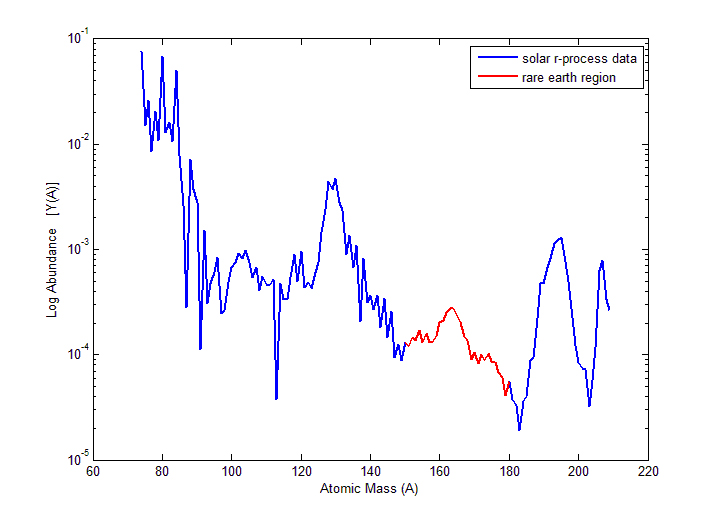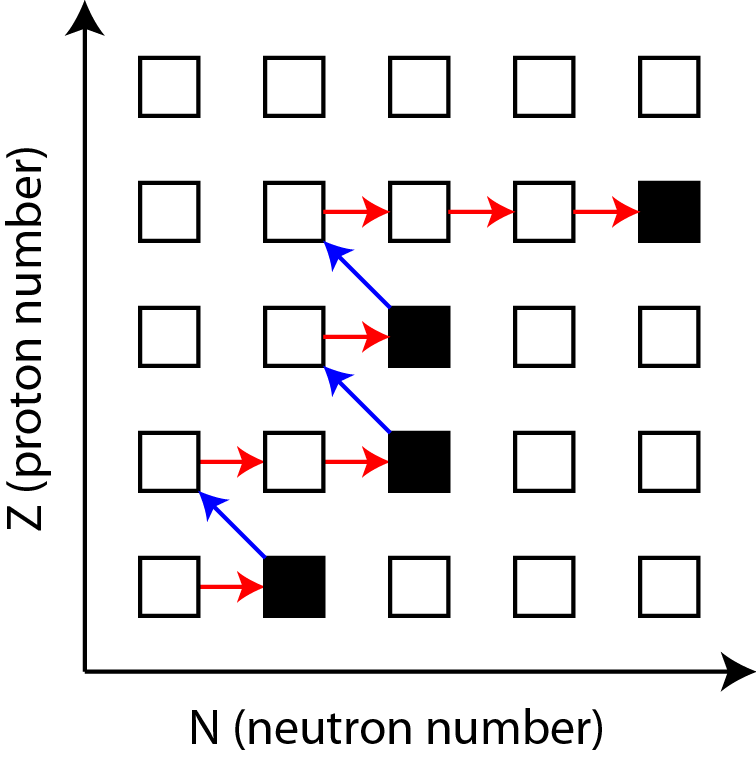Rapid neutron capture that occurs in astrophysical environments allowing for the production of heavy elements

Neutron captures are initially much faster than $\beta$-decays

Relative slowdown in the nuclear flow (right) produces peak structures in the observed abundances (left)

Astrophysical environment must produce a lot of free neutrons in order for this process to proceed

Aprahamian et al. (2018) • Horowitz et al. J Phys G 083001 (2019)

## Where can the $r$-process occur?

One possibility is in (rare?) supernovae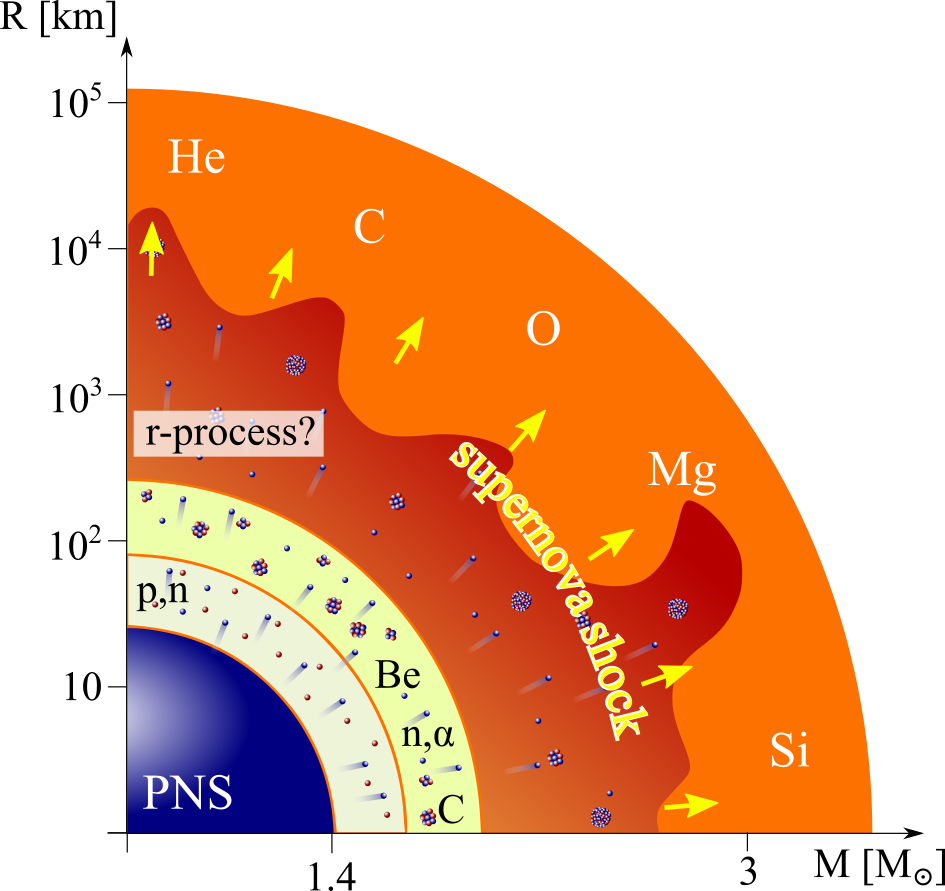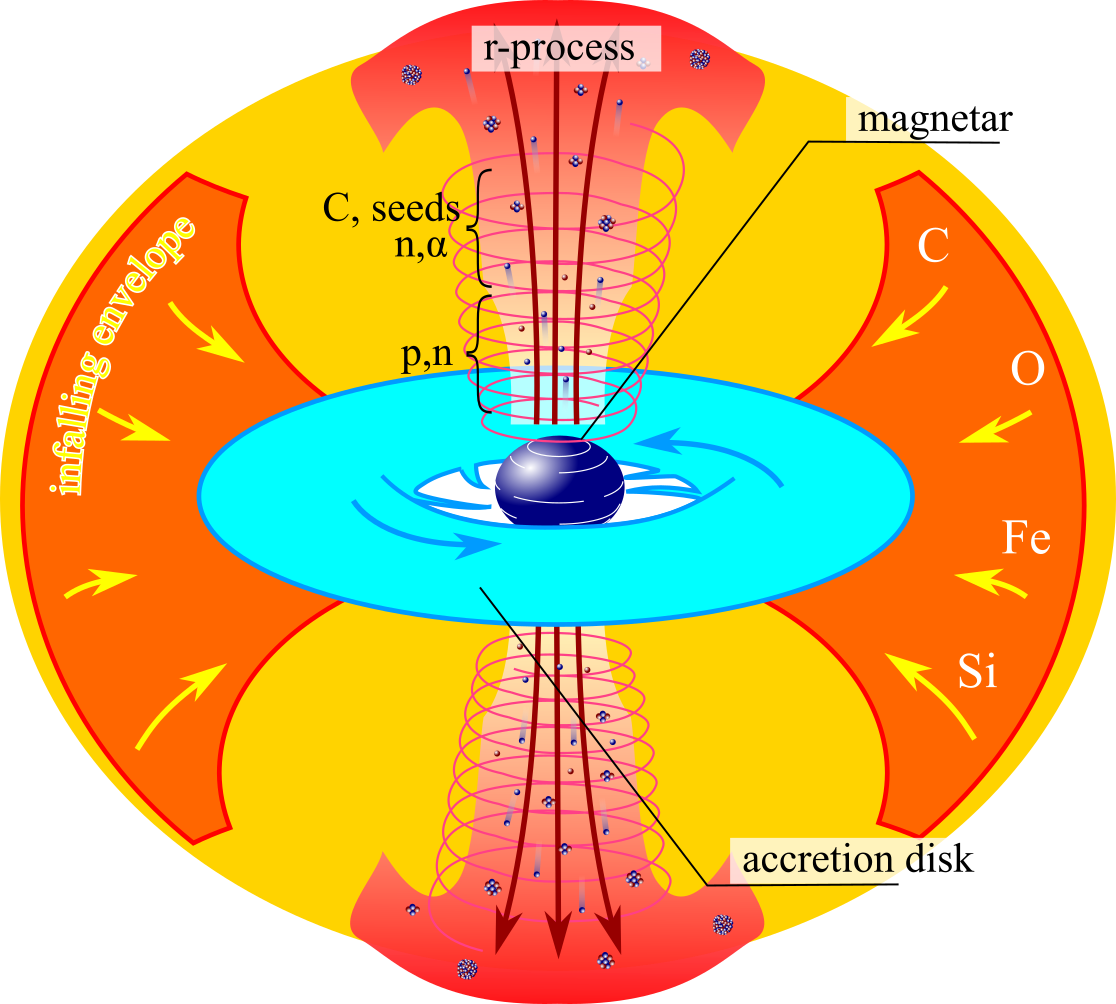For standard supernovae (left) neutrino physics still needs to be well understood

Jets in magnetorotational driven supernovae (right) may also provide the necessary conditions

Another option is the disk winds of collapsars - black hole forms after core collapse of a rapidly rotating star

Meyer ApJ (1989) • MacFadyen & Woosley ApJ (1999) • Figure by O. Korobkin

## Where can the $r$-process occur?

Another possibility is in compact object mergers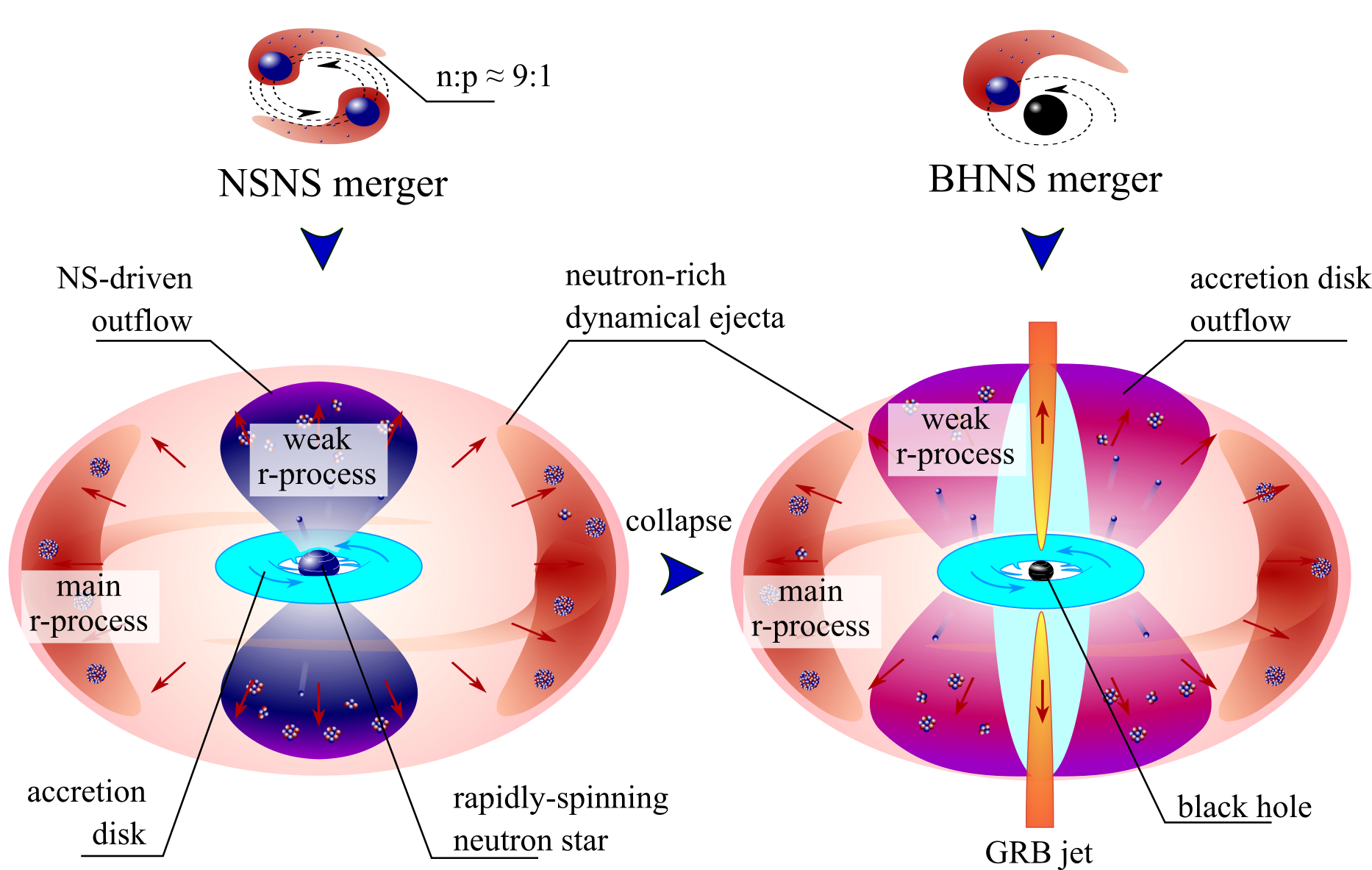A binary merger of neutron stars is an exciting possibility (some indirect evidence exists)

Another option is in the disk of a black hole neutron star binary

Lattimer & Schramm (1974) • Korobkin et al. (2012) • Figure by O. Korobkin

## How can we observe the $r$-process?

Gravitational waves are emitted by explosive events (can be detected by LIGO)

Electromagnetic signals may be detected from the radioactive decay of heavy nuclei

The problem with EM signals is that we can only detect them if the event is close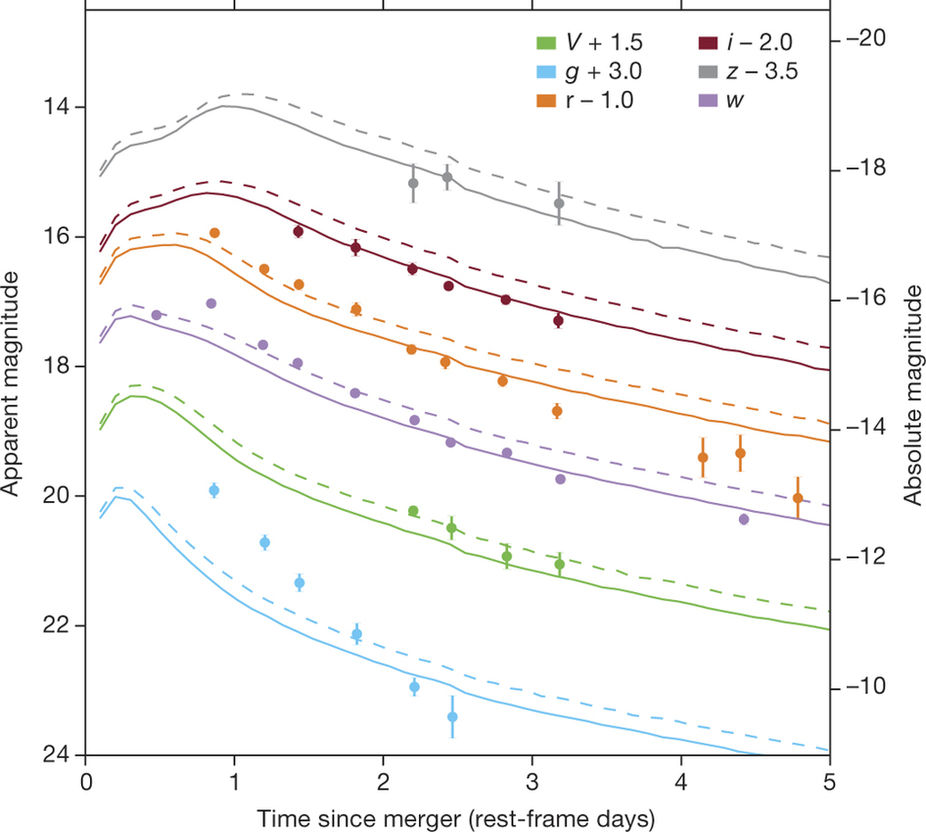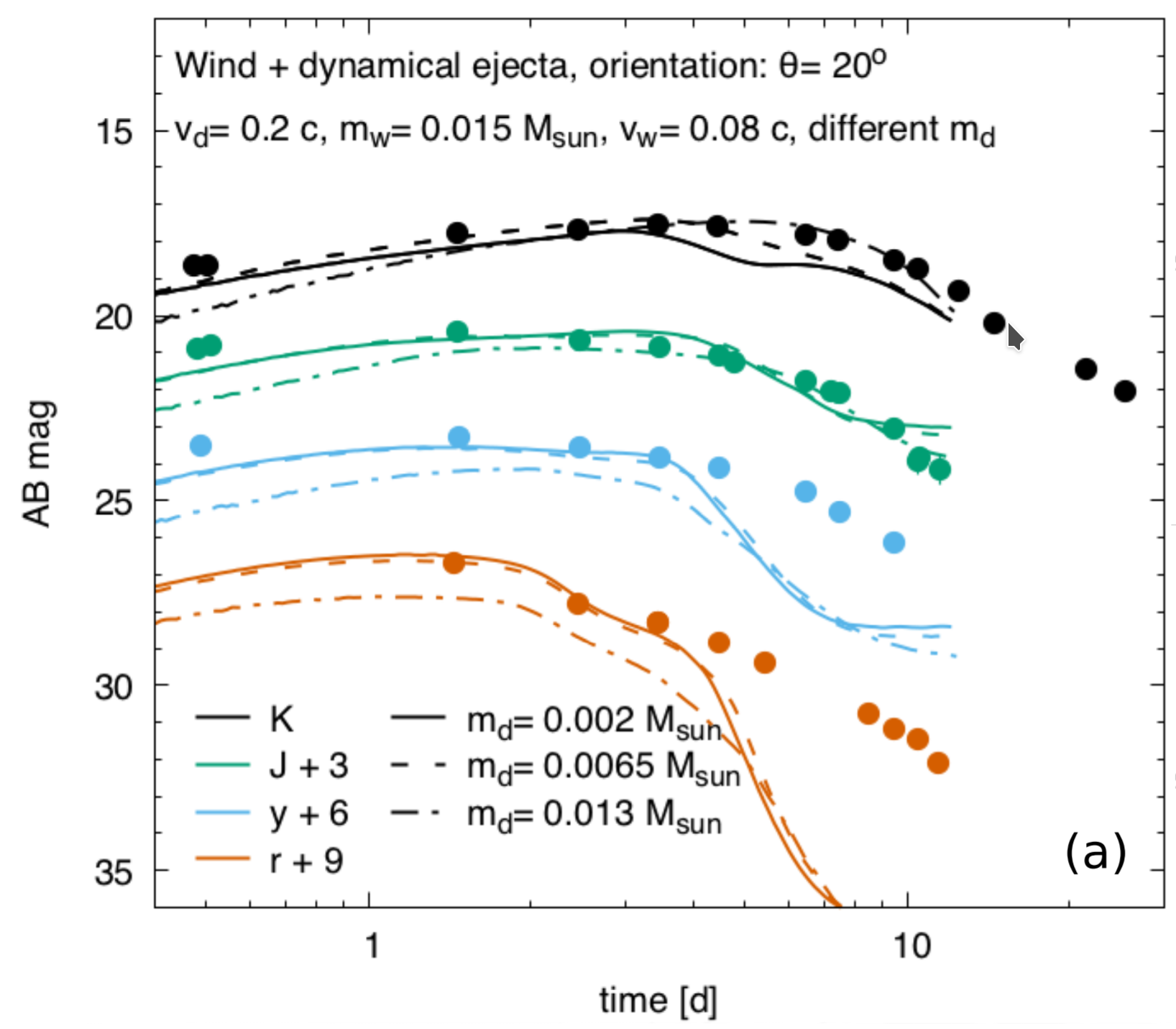(left) Low lanthanide fraction (right) high lanthanide fraction → major degeneracies in the model space

Arcavi et al. Nature 551 64 (2017) • Tanvir et al. ApJL 848 2 (2017)

## To understand the formation of the elements

Requires deep knowledge of a range of fields, including:

The theoretical modeling of astrophysical environments

Multi-messenger observations (gravitational waves, EM waves, etc.)

Nuclear theory predictions for exotic nuclei

Precision experiments to constrain nuclear theory

Data and observations are limited

We must be clever when deciphering what is going on with nucleosynthesis...

Especially if we want to find the fingerprints of heavy element formation!

## Nuclear physics efforts @ LANL have been focused on

Creating tools for the community to use to understand the $r$-process

Here's a few that will be featured today...

NEXUS

(A computational toolkit for synthesizing nuclear data & modeling relevant nuclear physics properties)

URSA: Unified Reaction Structures for Astrophysics

(A novel way to incorporate the latest nuclear data in astrophysical simulations)

PRISM: Portable Routines for Integrated nucleoSynthesis Modeling

(A state-of-the-art nuclear reaction network)

(A state-of-the-art decay network for modeling late-time behavior)

Open source releases are available / forth coming!

## Radionuclides in the $r$-process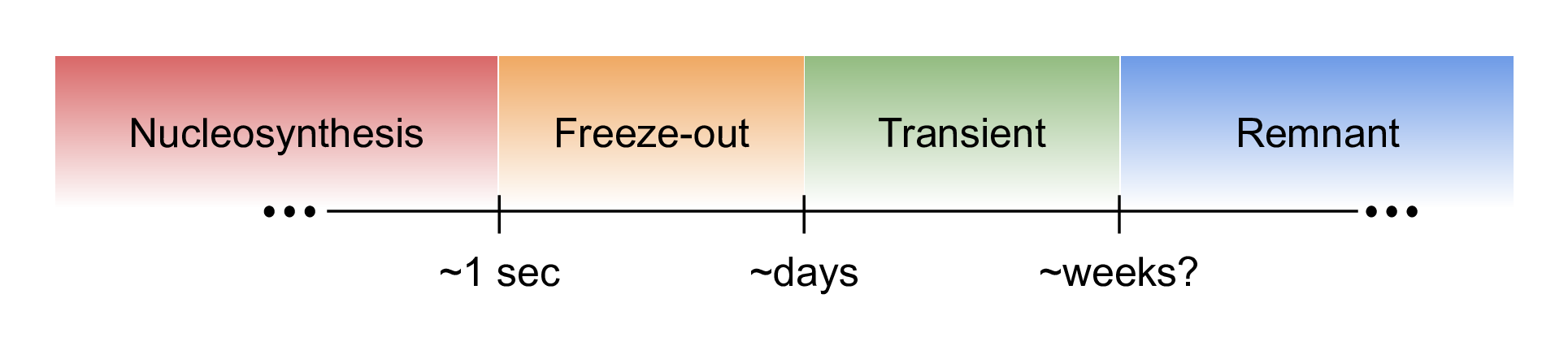While the nucleosynthesis of the $r$-process is short-lived (~1 second)

The creation of radionuclides spans half-lives from microseconds to near-stable

Different epocs have unique and interesting radioactive nuclei present along with associated signatures

Figure by Mumpower

## Nucleosynthesis epoch ($\tau \sim 1$ second)The most uncertain part of the $r$-process

How high up in mass number ($A$) can this process achieve?

We need reliable nuclear theory calculations to address such open questions... (NEXUS / URSA / PRISM)

Figure by Mumpower

## Novel description of $\beta$-decay: QRPA + HF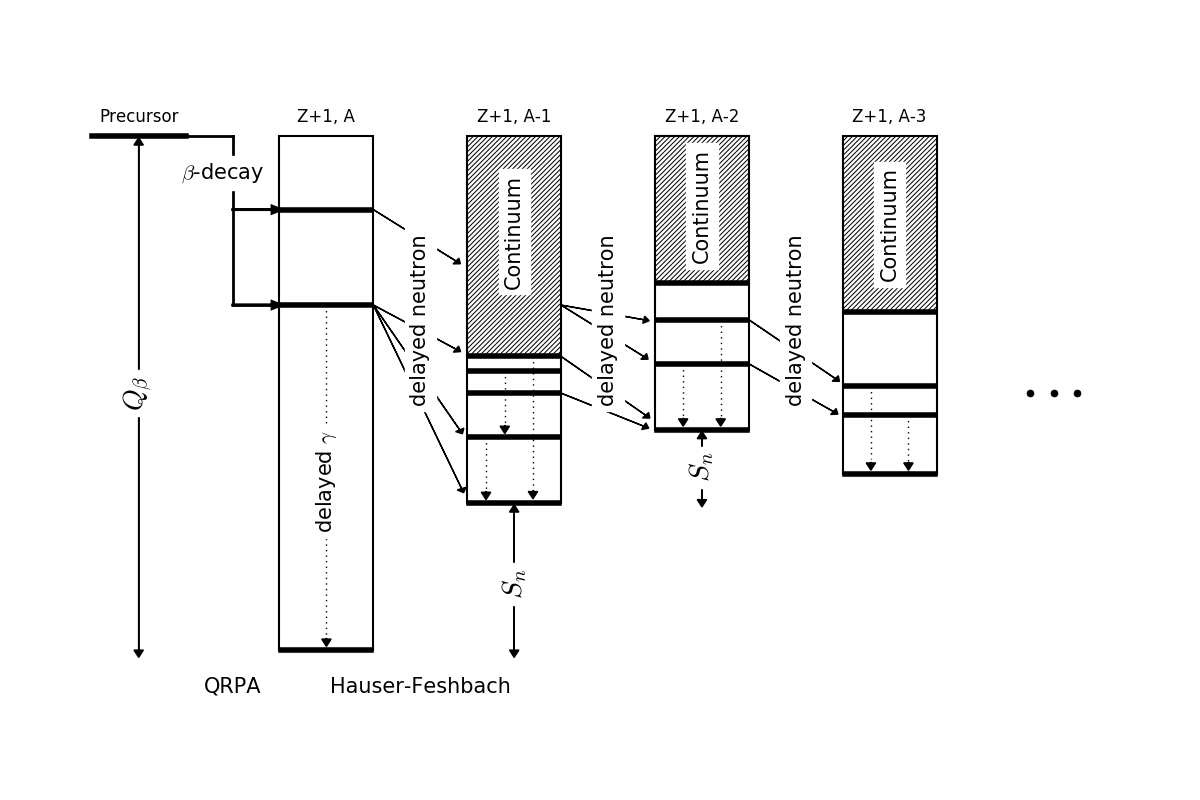Initial population from the $\beta$-decay strength function from QRPA

Follow the statistical decay via Hauser-Feshbach until all excitation energy is exhausted

Mumpower et al. PRC 94 064317 (2016) • Spyrou et al. PRL 117 142701 (2016)
Wu et al. PRL 118, 072701 (2017) • Mumpower et al. ApJ 869 1 (2018) • Möller et al. ADNDT 125 (2019)

## Average neutron emissionApply energy window method to the entire chart of nuclides

Problem with describing very neutron-rich nuclei

Mumpower et al. PRC 94 064317 (2016)

## Average neutron emission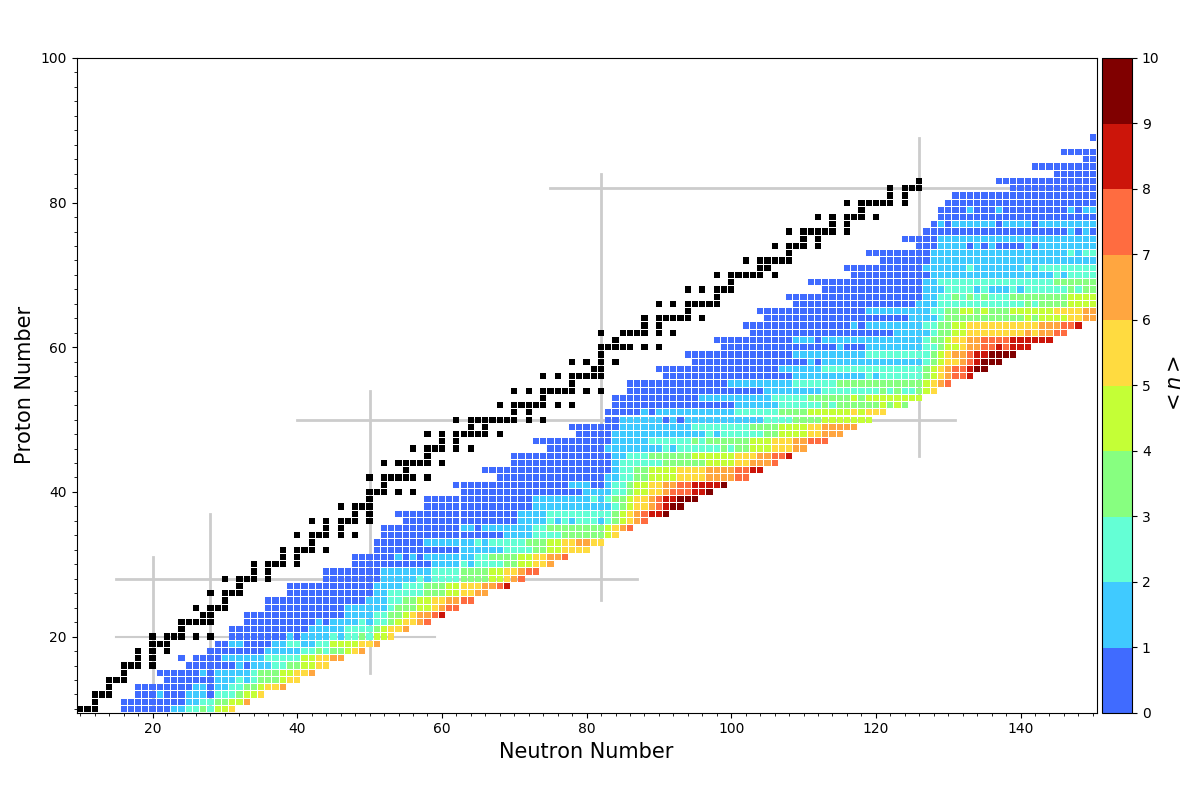Apply the QRPA+HF method to the entire chart of nuclides

Problem with neutron-rich nuclei goes away

Mumpower et al. PRC 94 064317 (2016) • Möller et al. ADNDT 125 (2019)

## $\beta$ -delayed fission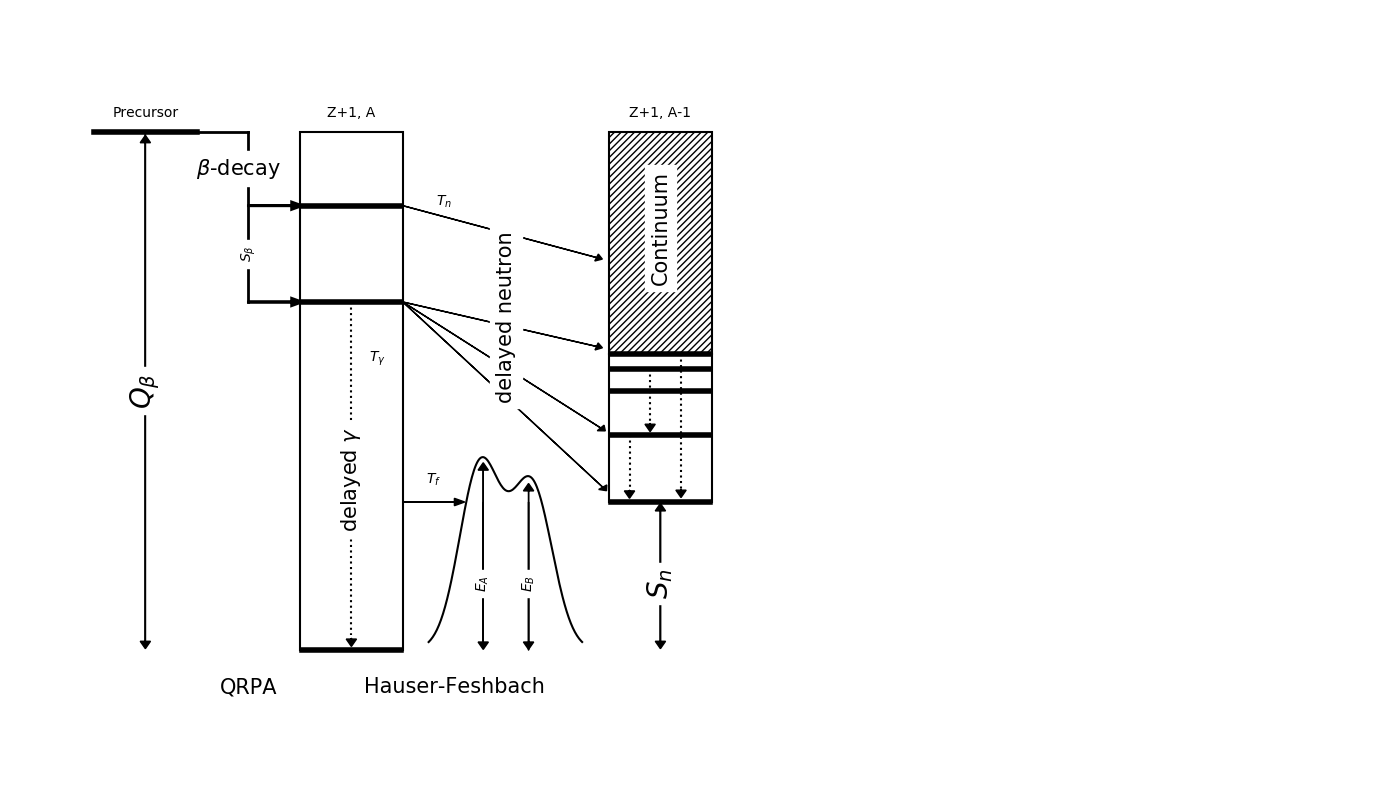We have recently extended our QRPA+HF model to describe $\beta$-delayed fission ($\beta$df)

Barrier heights from Möller et al. PRC 91 024310 (2015)

Assumes a Hill-Wheeler form for fission transmission

Mumpower et al. PRC 94 064317 (2016) • Spyrou et al. PRL (2016) • Möller et al. ADNDT 125 (2019)
Yokoyama et al. PRC (2019) • Mumpower et al. ApJ (2018)

## Multi-chance $\beta$ df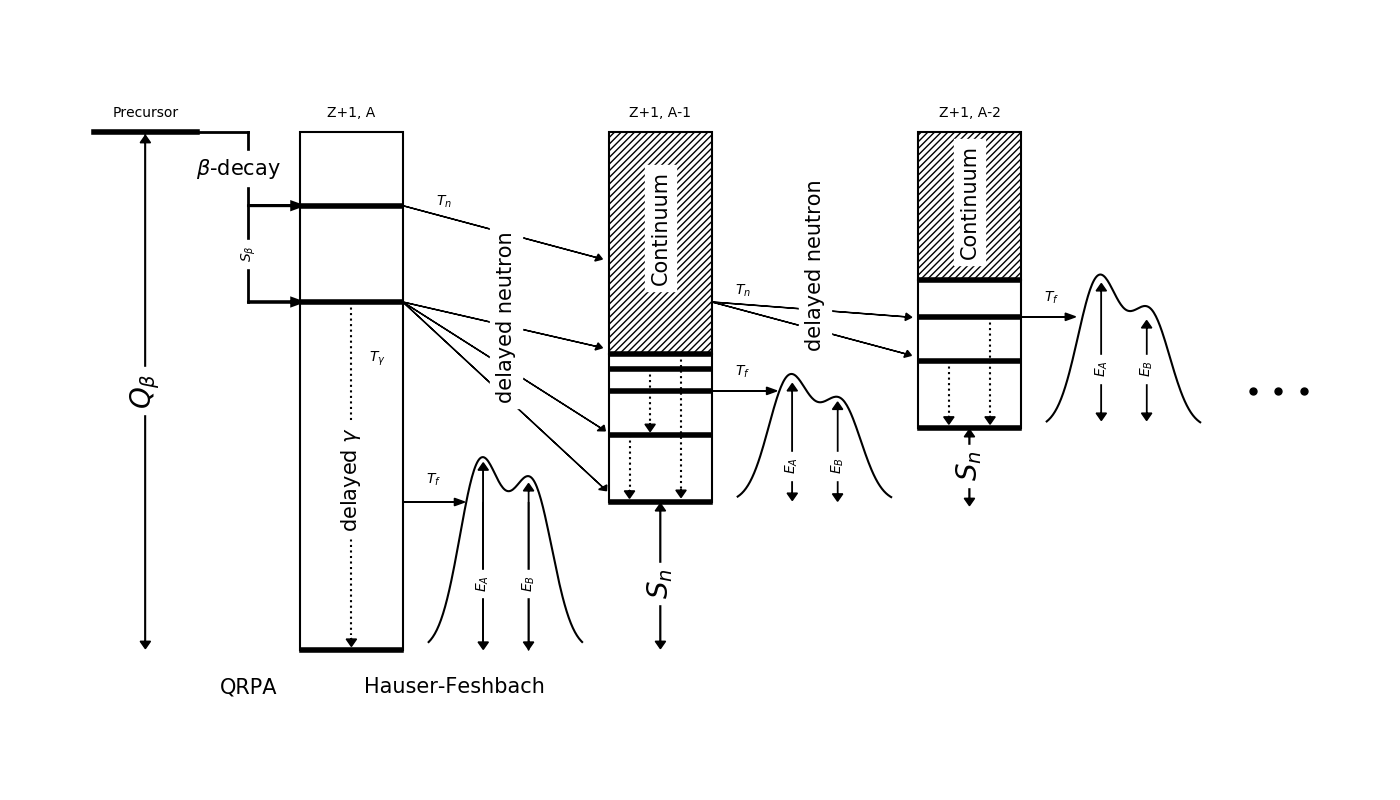Recall: Near the dripline $Q_{beta}$ ⇡ $S_{n}$ ⇣

Multi-chance $\beta$df: each daughter may fission

The yields in this decay mode are a convolution of many fission yields!

Mumpower et al. PRC 94 064317 (2016) • Spyrou et al. PRL (2016) • Möller et al. ADNDT 125 (2019)
Yokoyama et al. PRC (2019) • Mumpower et al. ApJ (2018)

## Cumulative $\beta$ df probability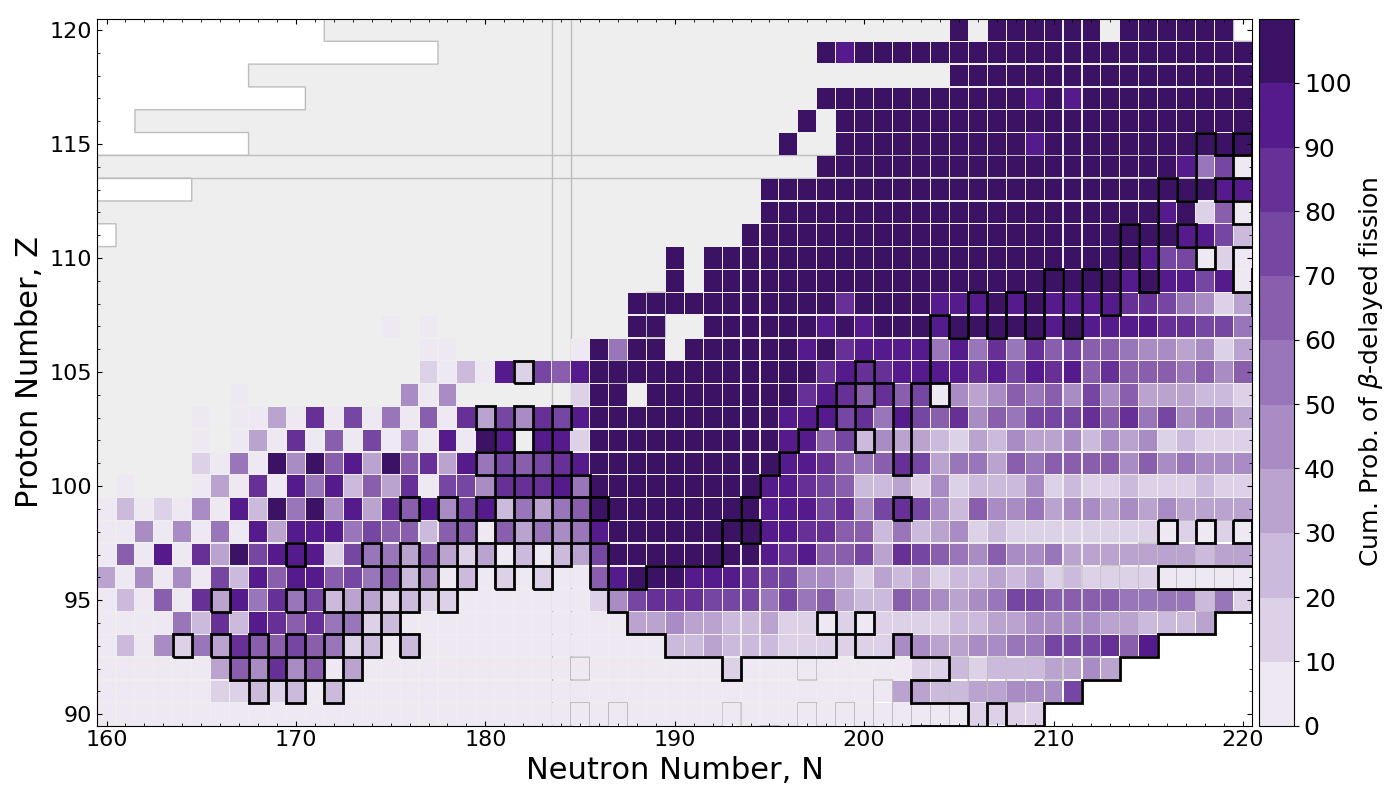$\beta$df occupies a large amount of real estate in the NZ-plane

Multi-chance $\beta$df outlined in black

Mumpower et al. ApJ 869 1 (2018)

## Superheavies in the $r$-processNetwork calculation of tidal neutron star merger ejecta

$\beta$df alone may prevent the pathway from neutron-rich nuclei to the island of stability

Thielemann et al. Zeitschrift fur Physik A Atoms and Nuclei 309 4 (1983) • Mumpower et al. ApJ 869 1 (2018)

## Fission can impact final abundances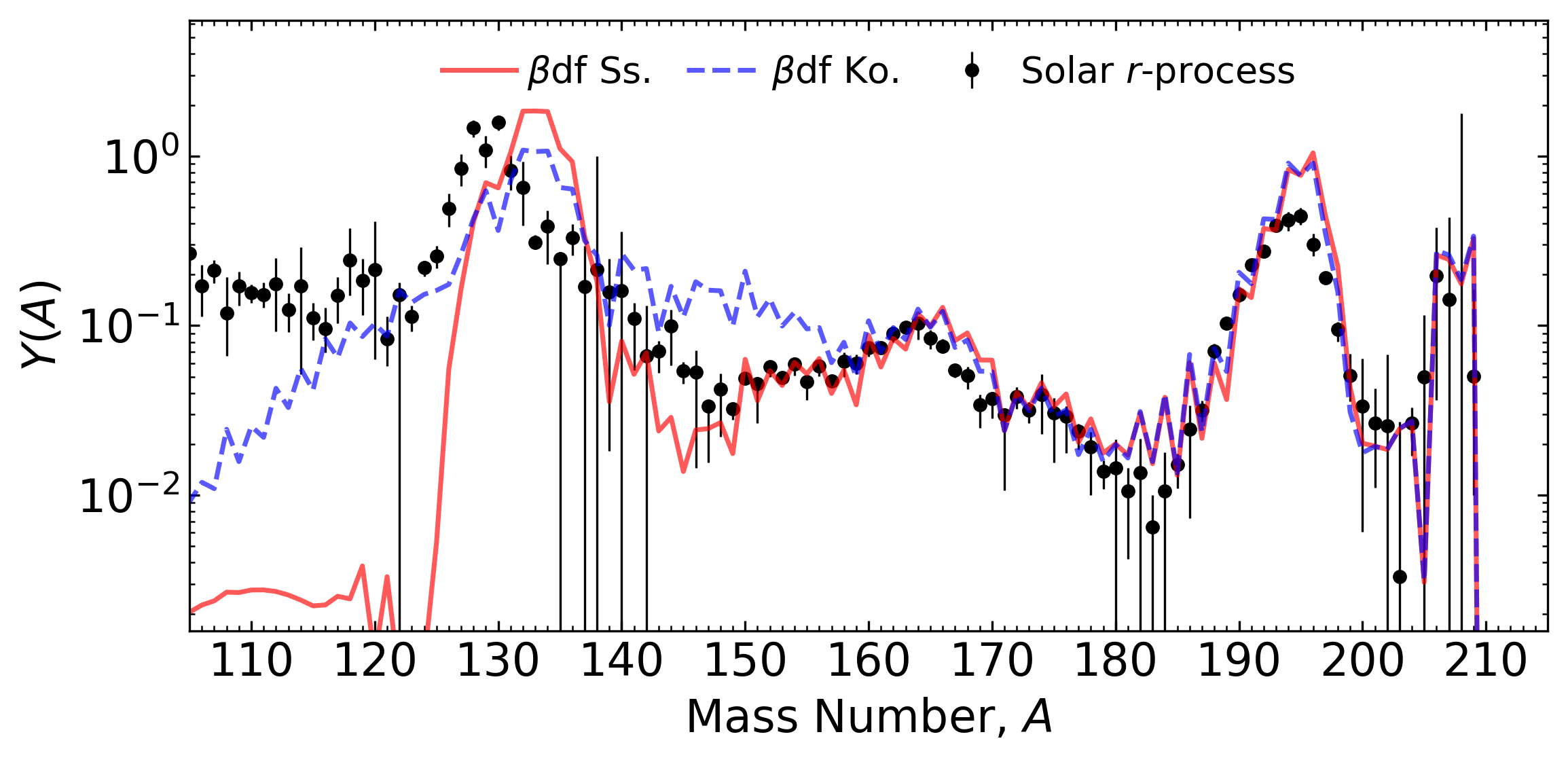Network calculation of tidal ejecta from a neutron star merger (FRDM2012)

$\beta$df can shape the final pattern near the $A=130$ peak

This is because of a relatively long fission timescale

Conclusion ⇒ we need a good description of fission yields to understand abundances near $A\sim130$.

Kodama & Takahashi (1975) • Shibagaki et al. ApJ (2016) • Mumpower et al. ApJ 869 1 (2018) • Vassh et al. J. Phys. G (2019)

## Nuclear fission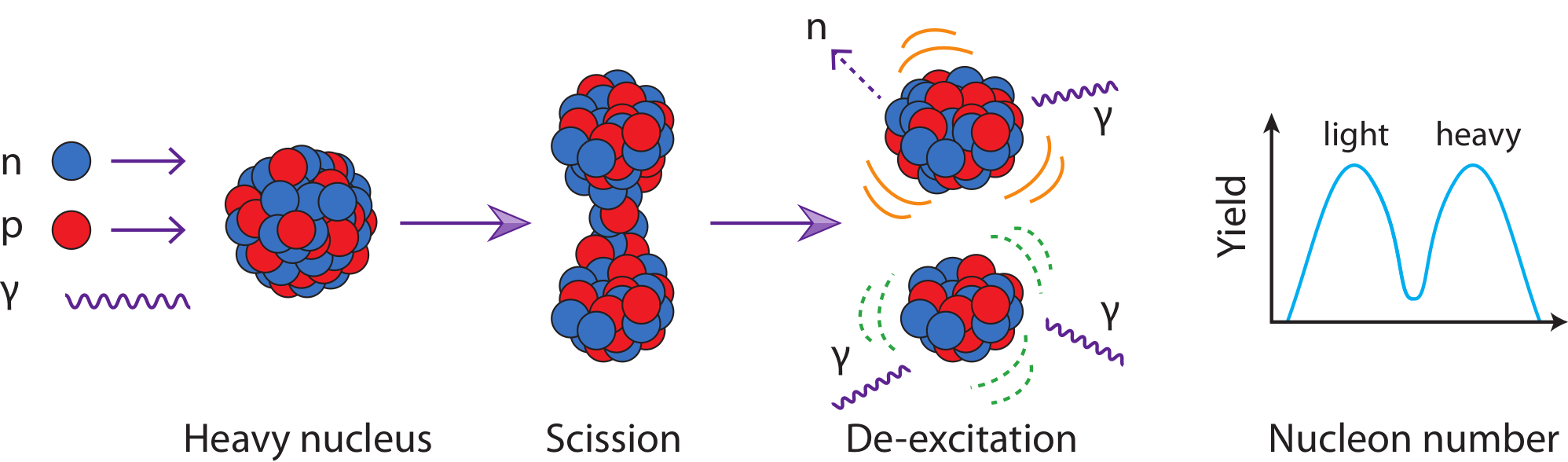We're also interested in describing the mass and charge yields that arise from nuclear fission

Fission is a complex process in which a heavy nucleus splits into lighter fragments

Fission rates and branching determine re-cycling (robustness)

Fragment yields place material at lower mass number; barriers determine hot spots

Large Q-value ⇒ impacts thermalization and therefore possibly observations

Responsible for what is left in the heavy mass region when nucleosynthesis is complete ⇒ "smoking gun"

Eichler et al. APJ (2015) • Goriely et al. EPJA (2015) • Holmbeck et al. ApJ 870 1 (2019) • Vassh et al. J. Phys. G (2019) • Figure by Mumpower

## FRLDM fission yields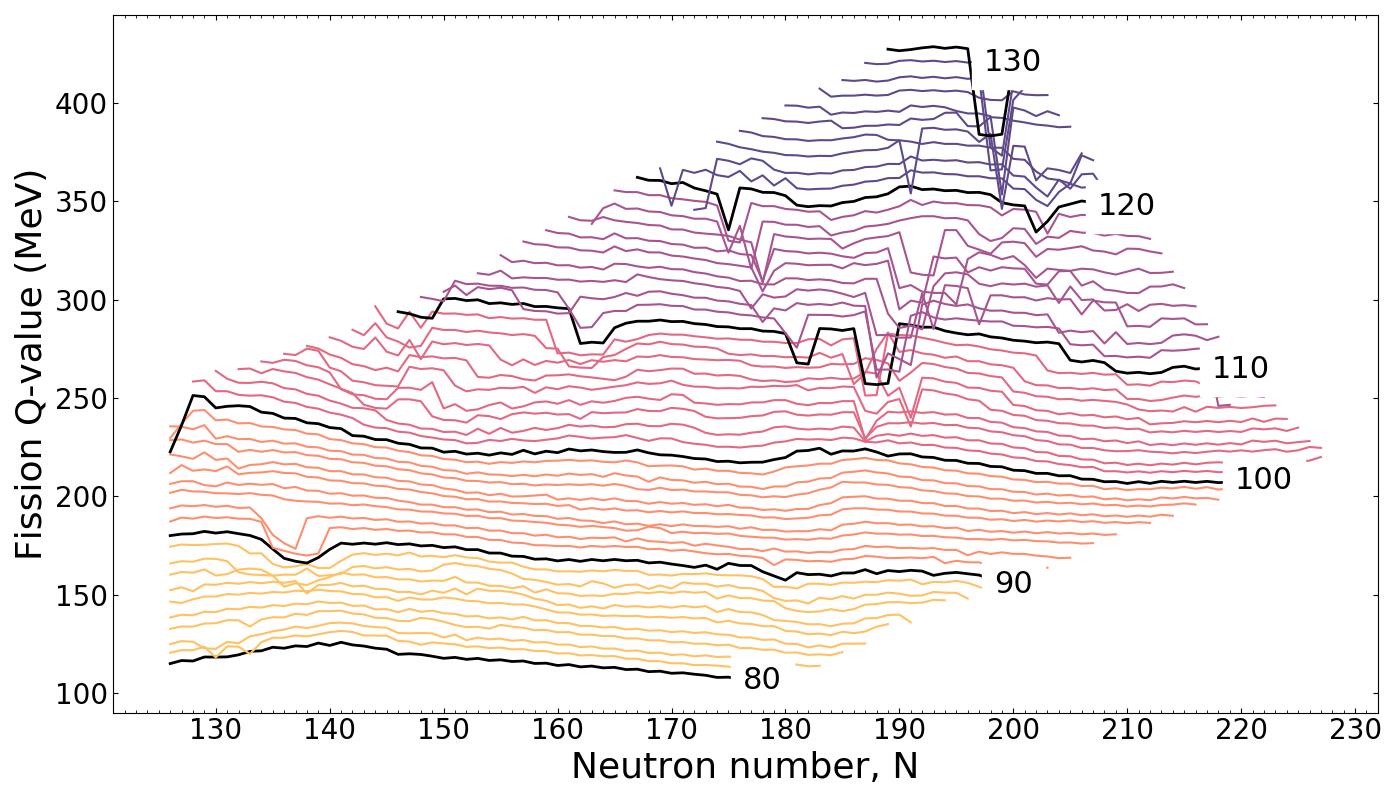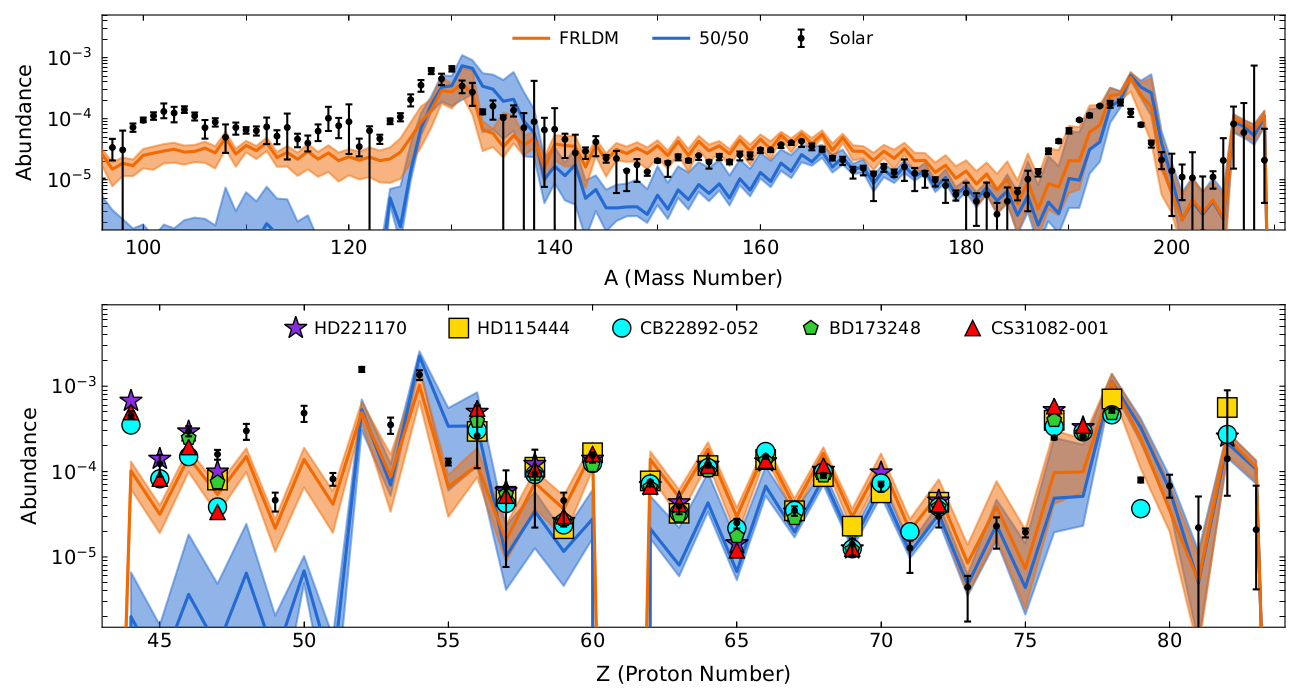Left panel: A complete description of fission yields suggests energy release depends mostly on fissioning system charge number

This is important for predictions of early time heating in the $r$-process

Right panel: Fission might be responsible for the extension of universality down to the precious metals

More complete observations with metal poor stars would be very helpful!

See Nicole's presentation on Thursday for more lanthanide & actinide signatures!

Mumpower et al. PRC 101 054607 (2020) • Vassh et al. ApJ 896 1 (2020)

## eFRLDM Results: $^{235}$U + n$_{\rm{therm}}$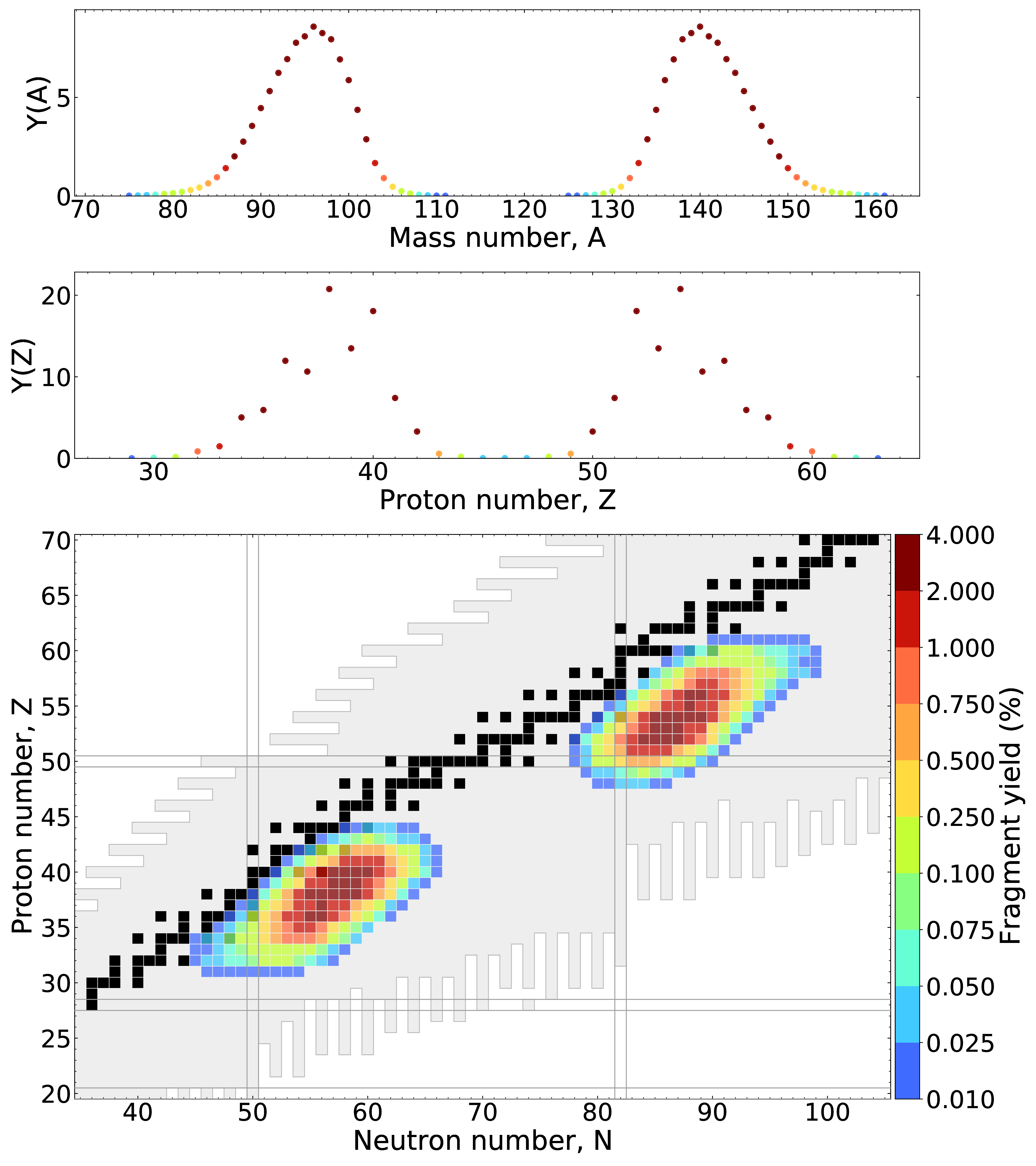We recently improved our fission modeling and can now describe Y(A), Y(Z) and Y(Z,A) simultaneously

Verriere et al. PRC 100 024612 (2019) • Verriere & Mumpower PRC 103 034617 (2020)

## eFRLDM Results: $^{235}$U + n$_{\rm{therm}}$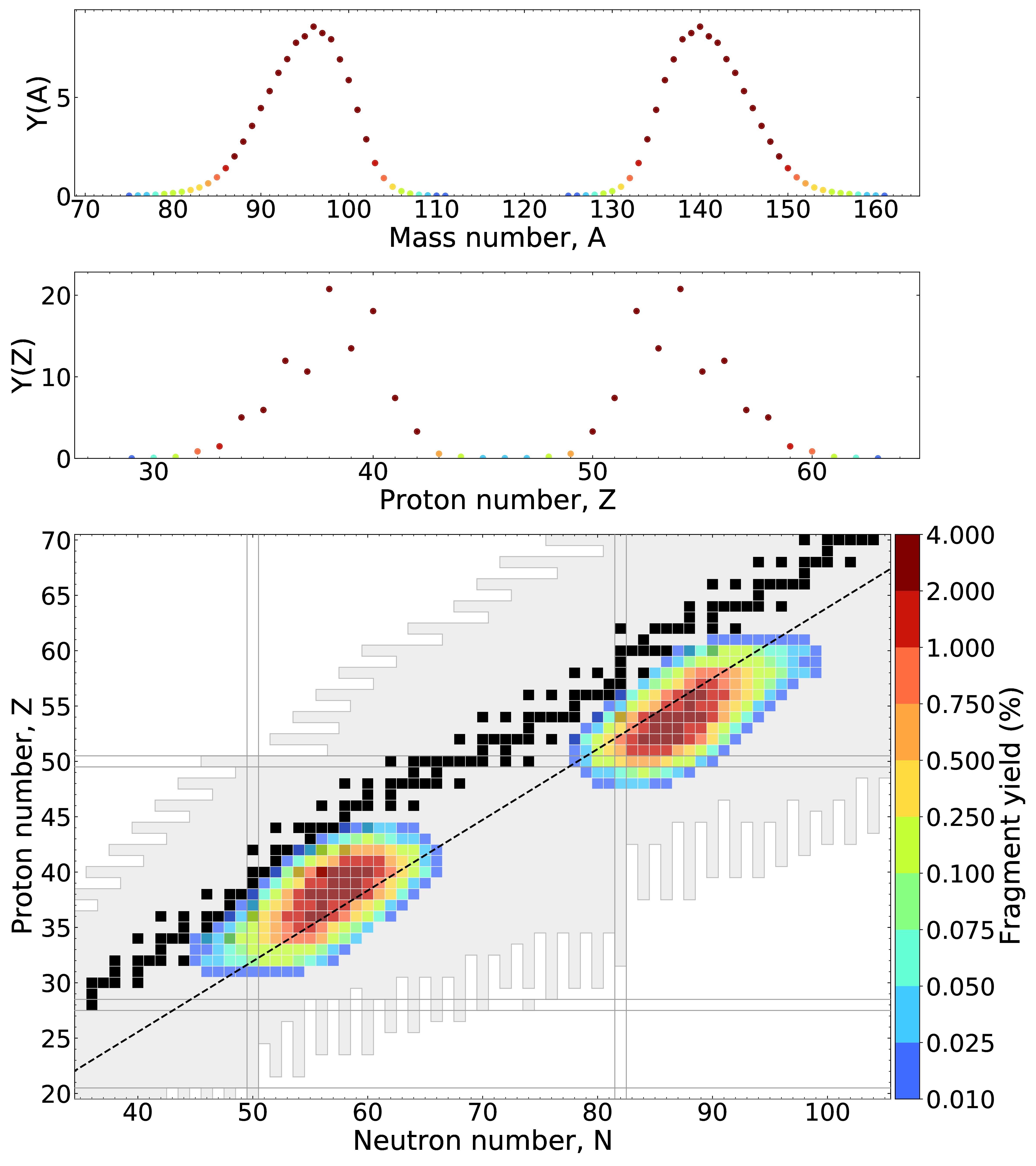Fragment yields no longer follow unchanged charge distribution (UCD) assumption (Blacked dashed line)

Verriere et al. PRC 100 024612 (2019) • Verriere & Mumpower PRC 103 034617 (2020)

## eFRLDM Results: $^{235}$U + n$_{\rm{therm}}$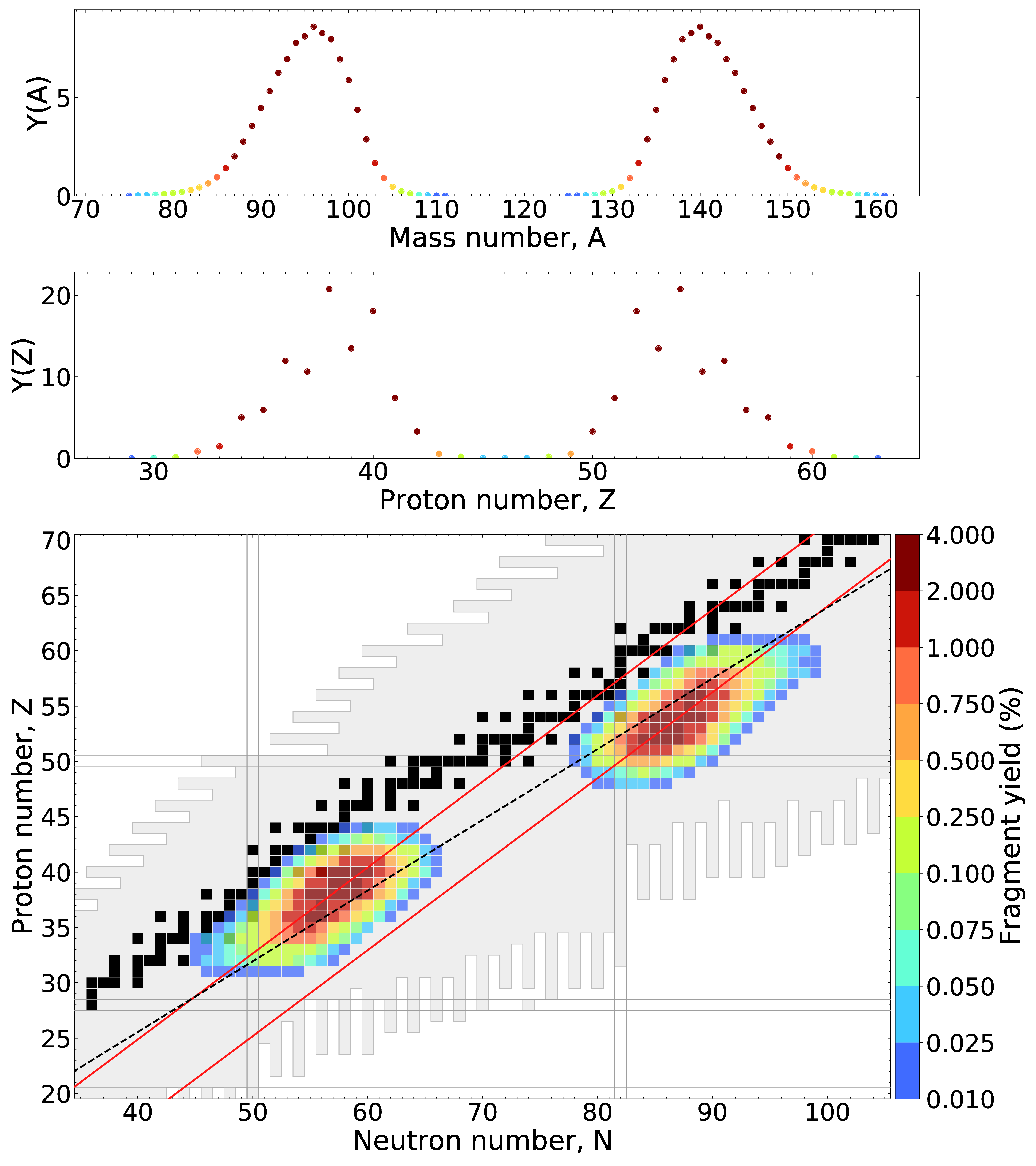Charge polarization offset predicted and in agreement with experimental measurements (red lines)

Verriere et al. PRC 100 024612 (2019) • Verriere & Mumpower PRC 103 034617 (2020)

## An example case: $^{258}$Fm ($Z=100$)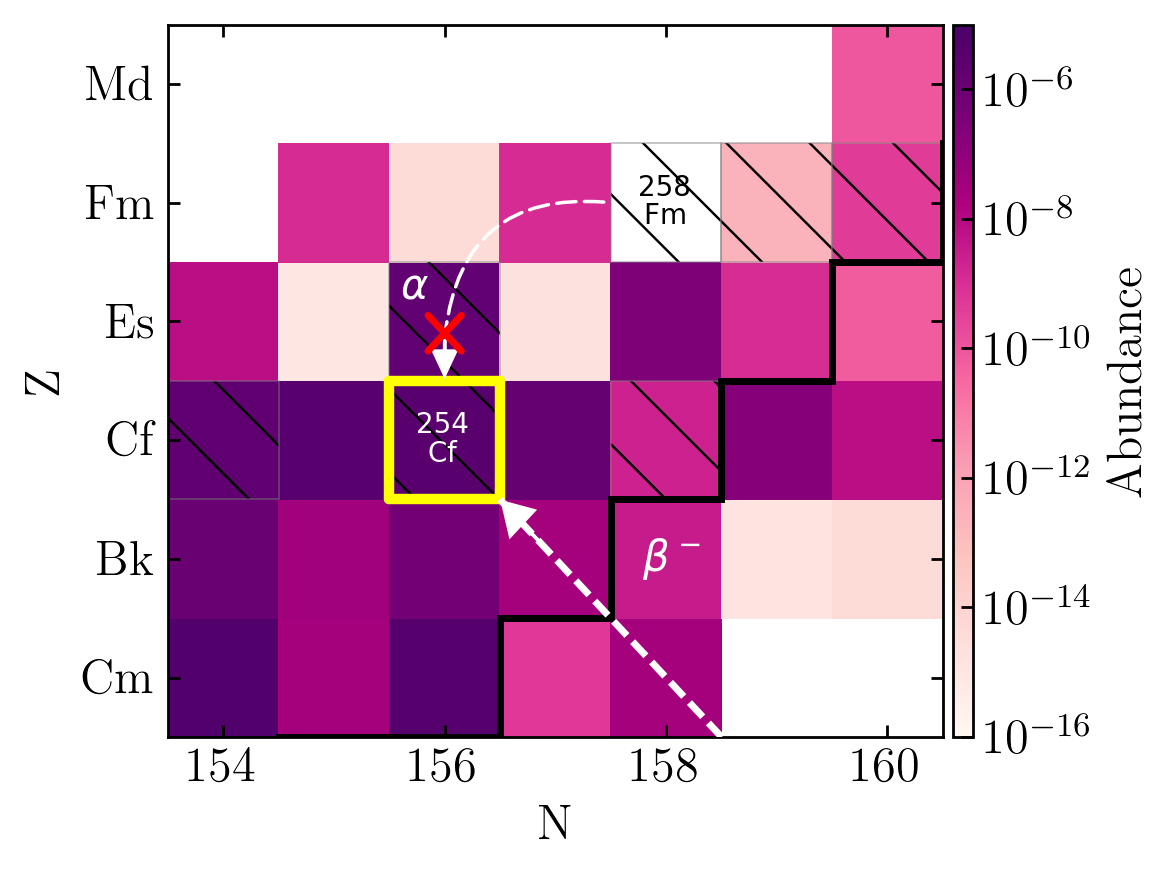$^{258}$Fm is a nucleus with a half-life of several hundred microseconds (populated in the nucleosynthesis epoch)

$^{258}$Fm has a small, but potentially non-trivial $\alpha$-decay branching to $^{254}$Cf; it primarily spontaneously fissions

To fully understand the potential implications of $^{254}$Cf production we need to have better measurements of $^{258}$Fm

We'll return to the importance of $^{254}$Cf soon (trasient epoch)...

Zhu et al. ApJL 863 2 (2018)

## Freeze-out epoch ($\tau \sim$ seconds to days)Nuclear transmutations are better constrainted; but we still lack information on excited states & particle spectra

Longer lived isomers are potentially populated and interesting ('astromers')

Some nuclei are strong contributors to the radioactive heating

A decay network is ideal for describing the physics of this timescale... (PRISM / JADE)

Figure by Mumpower

## Isochronic Evolution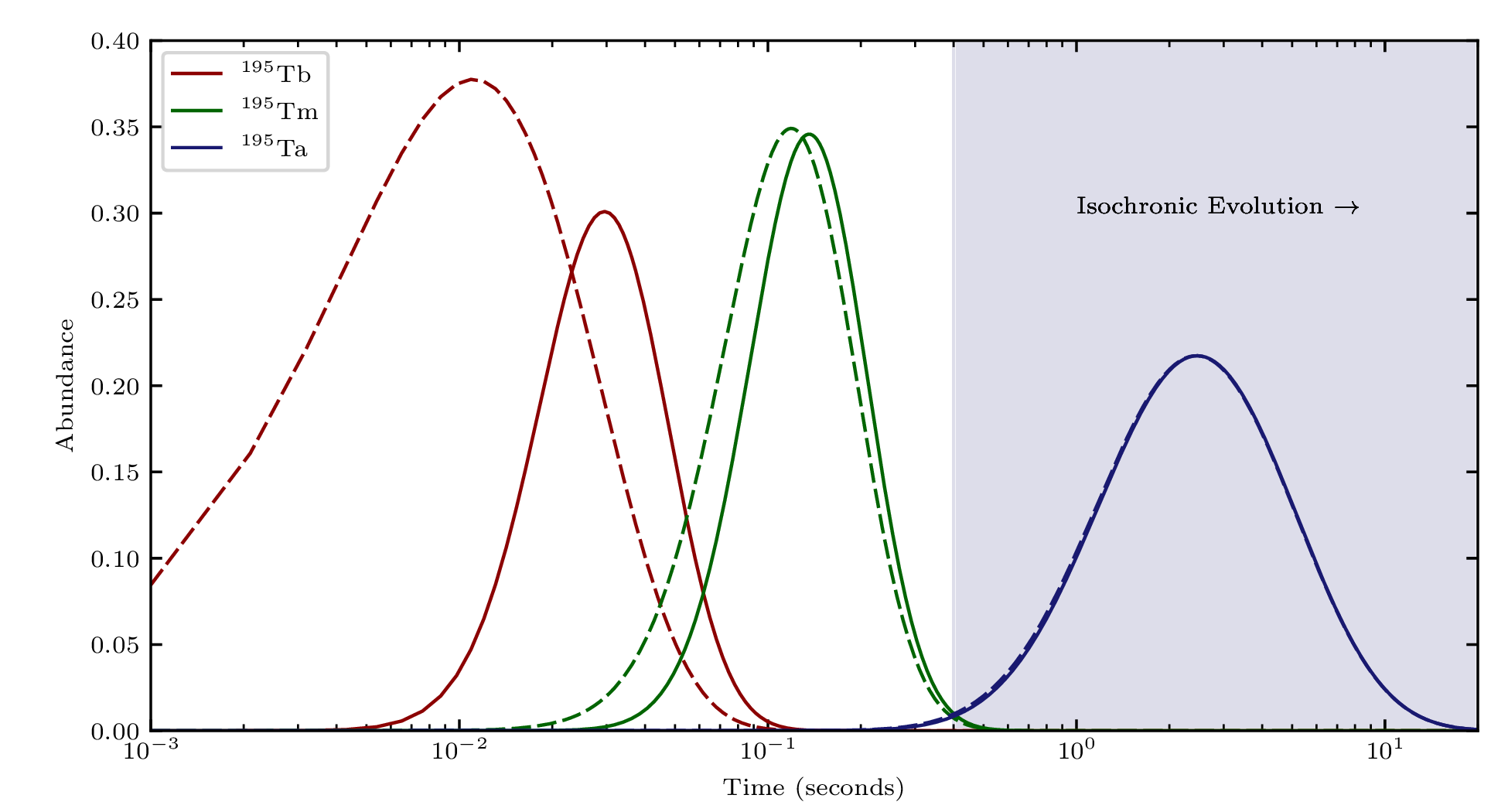If you wait long enough the evolution of abundances is isochronic

This means: two different distributions (solid vs dashed) early on later converge to the same curve; memory of initial state is lost

The bulk of r-process ejecta will undergo such an evolution; fission complicates this picture...

Sprouse et al. submitted (2021)

## Astrophysically metastable nuclear isomers (astromers)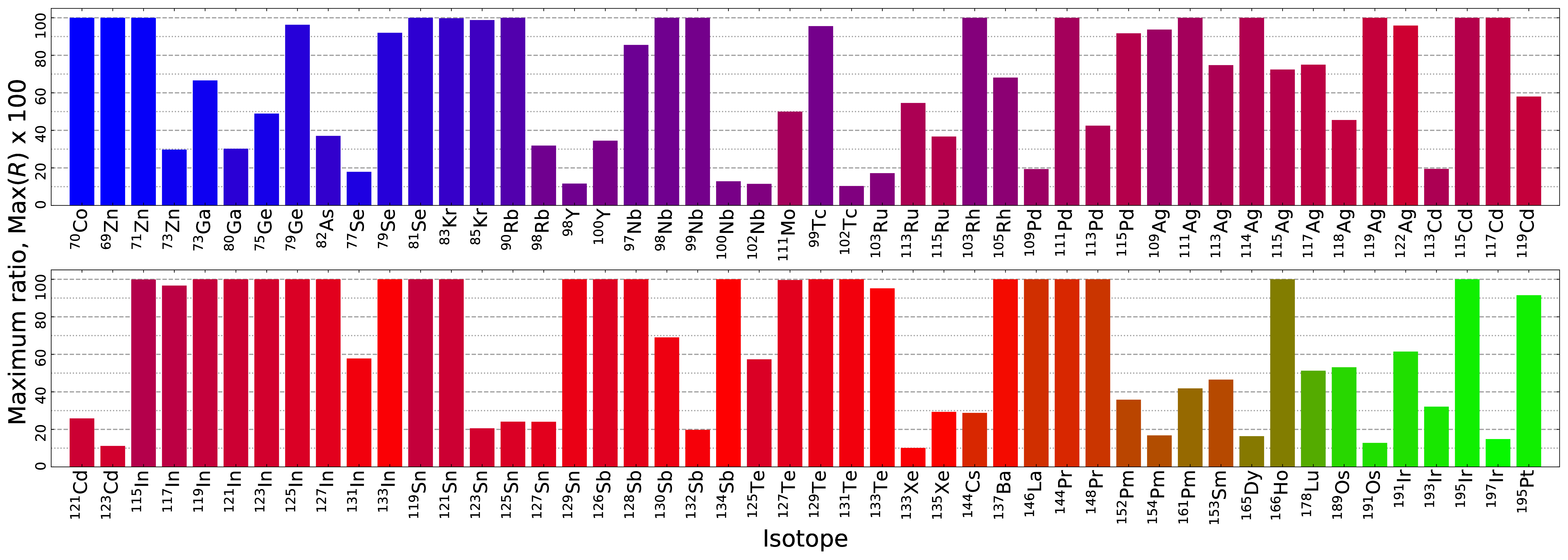Isomers are dynamically populated in the $r$-process

Their contributions to heating, light curves and observations can be quantified

Work pioneered by LANL postdocs: G. W. Misch (see Friday's presentation) & T. M. Sprouse

Nuclear databases are incomplete - there are certainly even more populated in the $r$-process!

Misch et al. ApJS 252 2 (2021) • Misch et al. ApJL 913 L2 (2021) • Misch et al. Symmetry 13 1831 (2021)

## Astrophysically metastable nuclear isomers (astromers)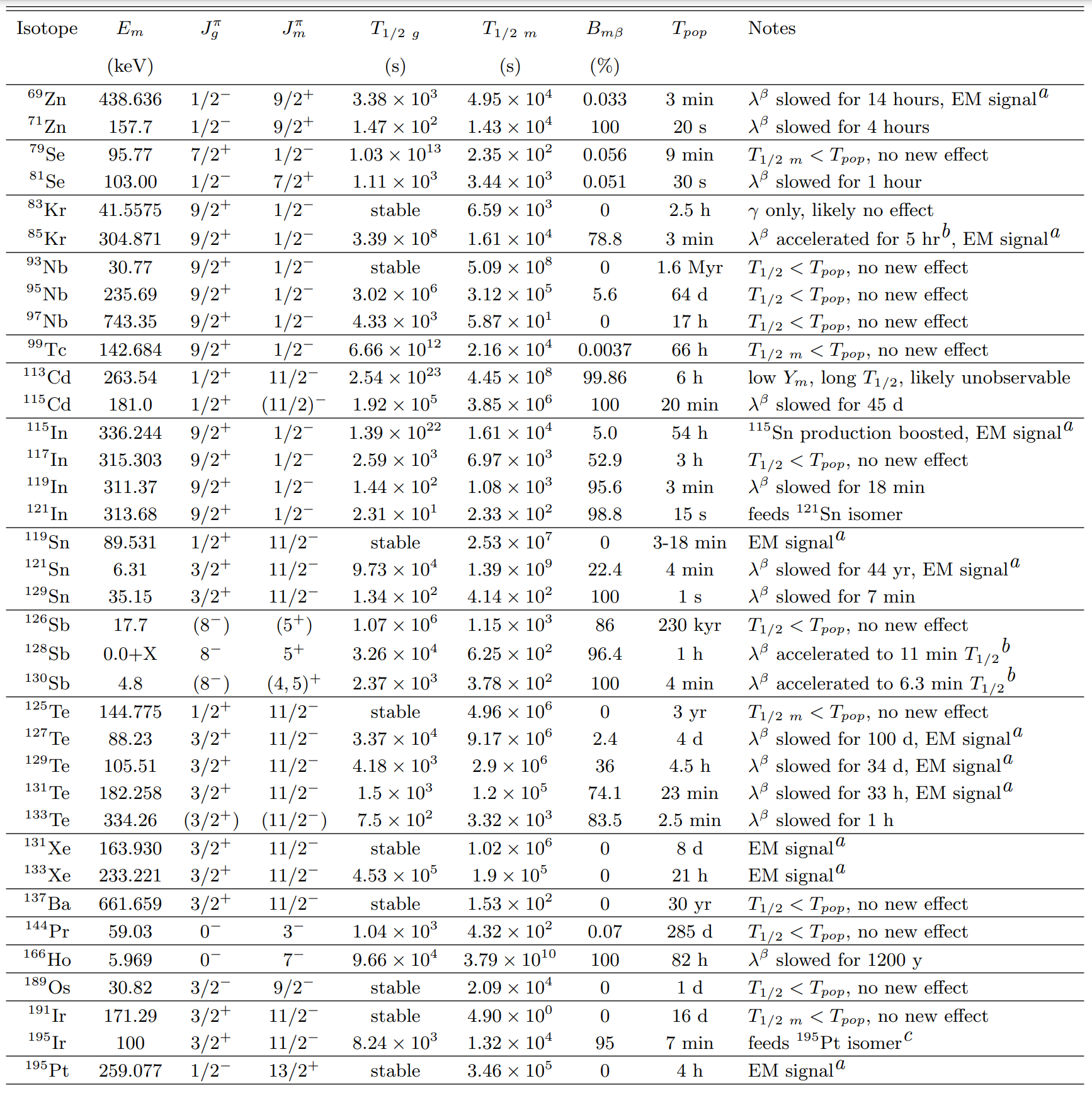A wide variety of nuclei are found with unique signatures

Misch et al. ApJS 252 2 (2021) • Misch et al. ApJL 913 L2 (2021) • Misch et al. Symmetry 13 1831 (2021)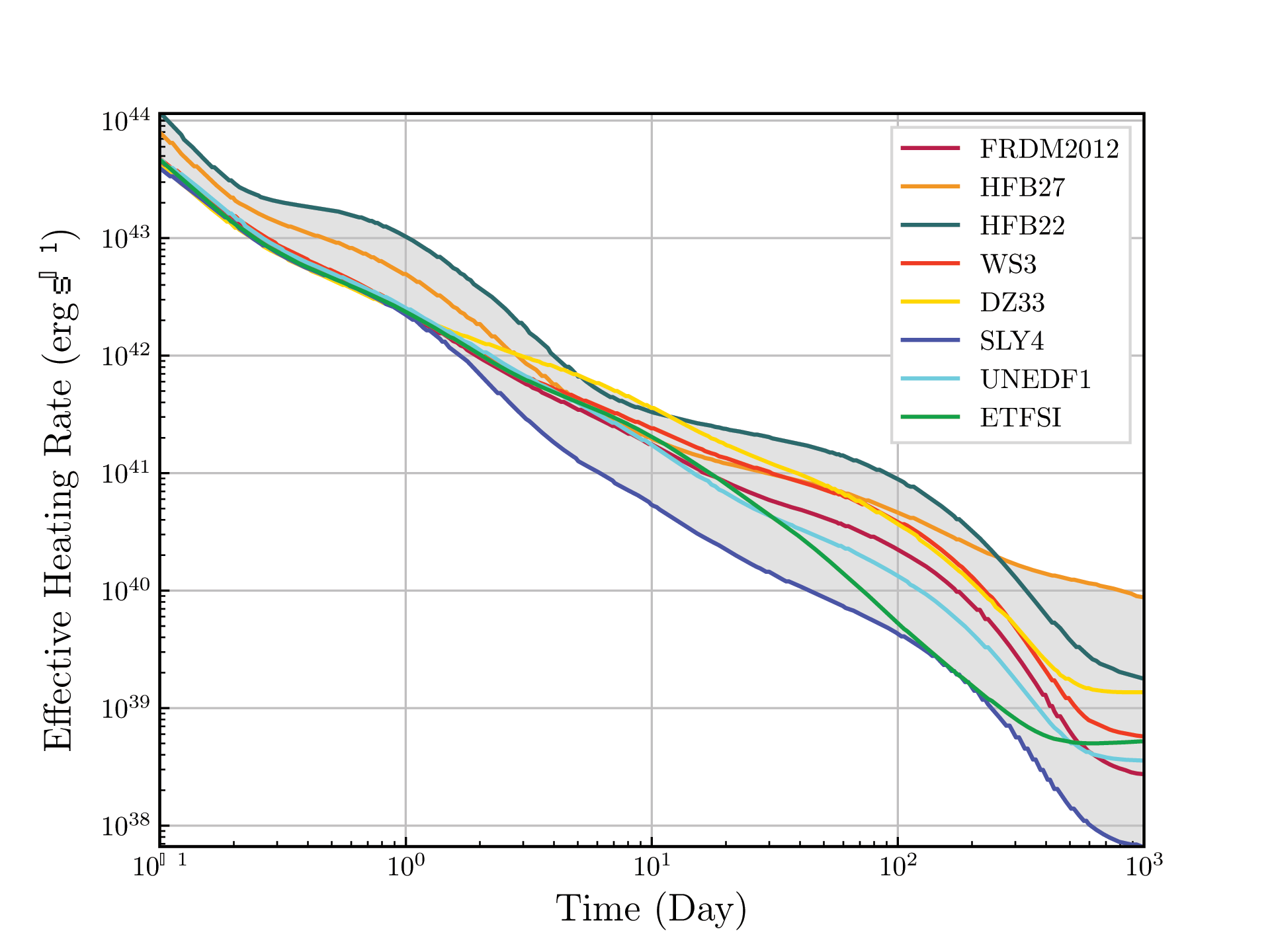Variation in radioactive heating from uncertainties in nuclear binding

Interesting nuclei can control energy release on characteristic timescales... e.g. I, La, Rf isotopes
(see Kelsey's presentation)

Zhu et al. ApJ 906 94 (2021) • Barnes et al. ApJ 918 44 (2021) • Lund et al. in prep. (2021)

## Transient epoch ($\tau \sim$ days to weeks?)Observationally interesting time period for a nascient $r$-process event

We must blend astrophysical simulations, nuclear physics and atomic physics to describe observables

Nebular phase requires non-LTE physics (hard!)

Figure by Mumpower

## $\gamma$-rays from mergers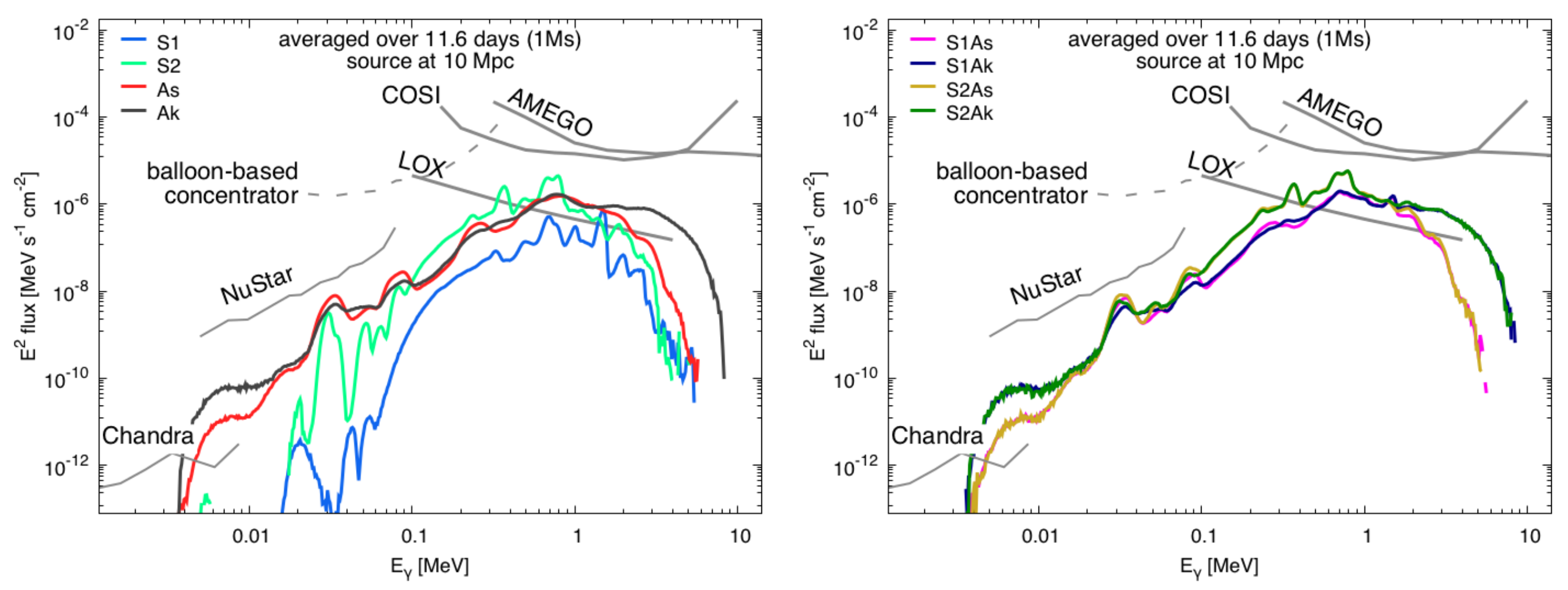If the $r$-process source is very close we might disentangle different compositions (colored lines)

Signatures of major emitters can come through (many second peak elements: e.g. isotopes of I, Sb, Sn)

Korobkin et al. ApJ 889 2 (2020)

## Fission $\gamma$-rays from mergers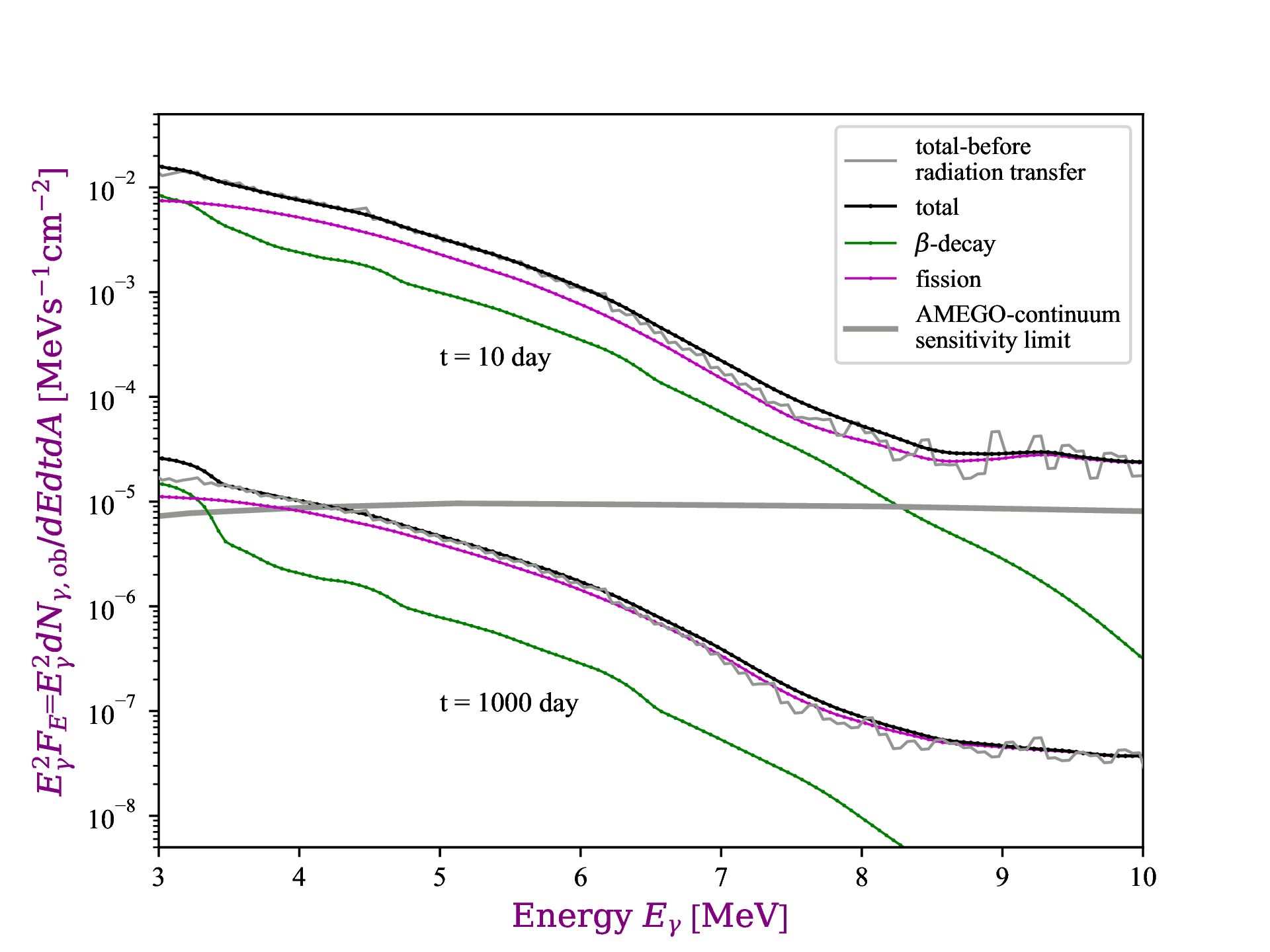$\gamma$-rays from fission have a distinct (higher energy) spectra

Spectra is smooth; cannot detect presence of individual radionuclides

It is however indicative of fission occuring (no other process can create such high energy $\gamma$'s)

Wang et al. ApJL 903 1 (2020)

## Returning to $^{254}$Cf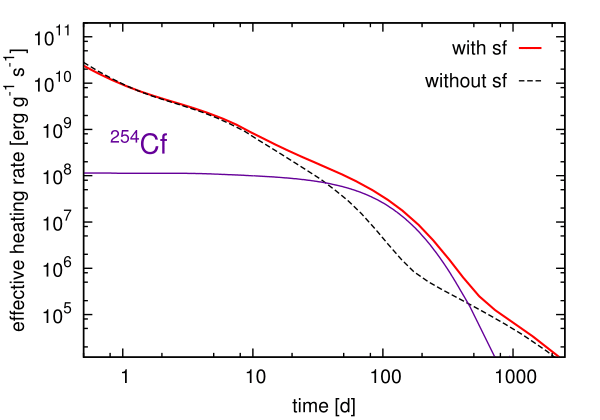Can we prove that all of heavy elements nucleosynthesis has occurred in an event?... Maybe!

The spontaneous fission of $^{254}$Cf can be a primary contributor to nuclear heating at late-time epochs

The $T_{1/2}\sim 60$ days; found from nuclear weapons testing

Baade et al. PASP (1956) • Conway et al. JOSA (1962) • Wanajo et al. ApJL (2014) • Y. Zhu et al. ApJL 863 2 (2018)
Vassh et al. J. Phys. G (2019)

## Production of $^{254}$Cf(Z=98)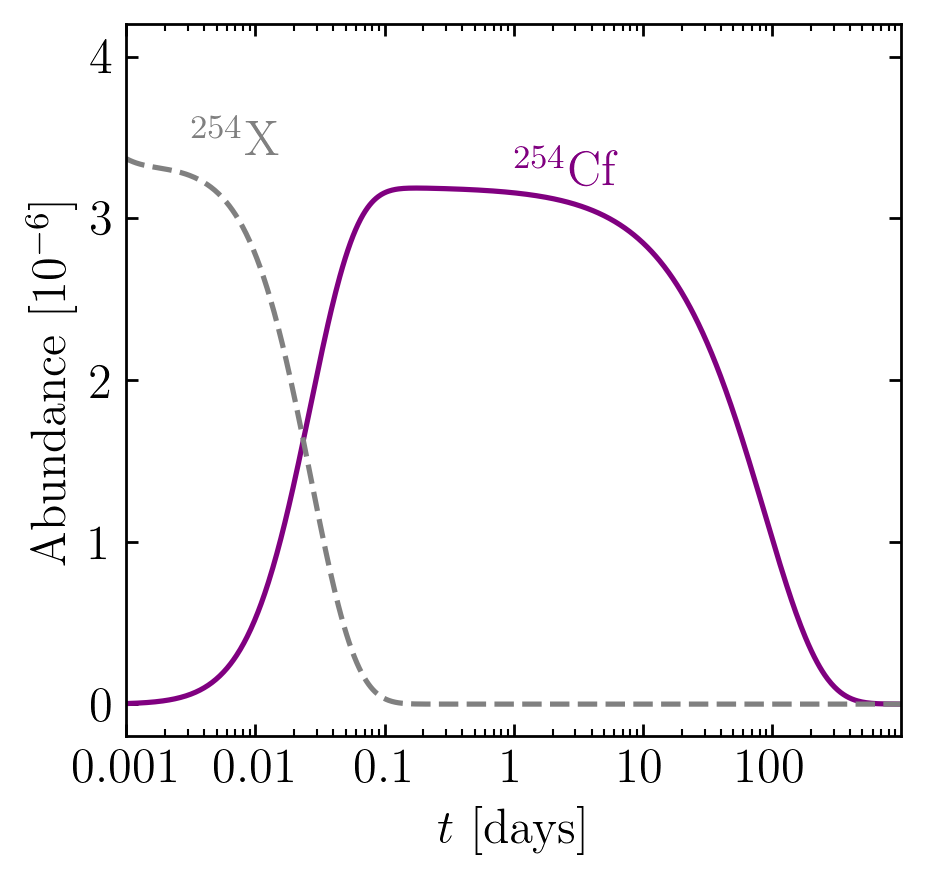Primary feeder seems to be from $\beta$-decay

Production of this nucleus been explored over a range of nuclear models; some high - some low

Remains to be seen if we can disentangle from other energy sources (e.g. puslar or accreetion fallback)

Wanajo et al. (2014) • Y. Zhu et al. ApJL 863 2 (2018) • Vassh et al. J. Phys. G (2019) • Wollaeger et al. ApJ (2019)

## Observational Impact of Californium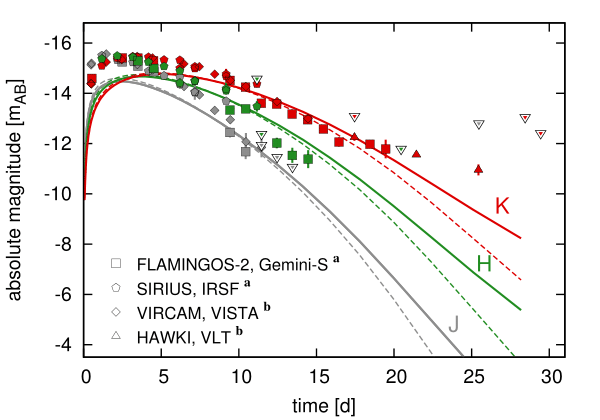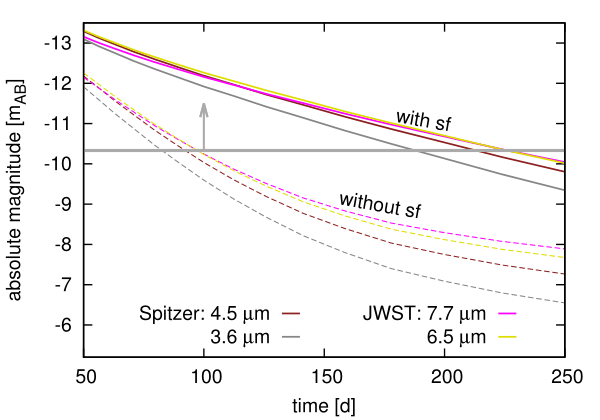Both near- and middle- IR are impacted by the presence of $^{254}$Cf

Late-time epoch brightness can be used as a proxy for heavy element nucleosynthesis

Future JWST mission could detect $r$-process event out to 250 days with the presence of $^{254}$Cf

This also has implications for compact object merger morphology...

Other nuclei remain interesting (e.g. $^{260}$Md with $T_{1/2} \sim 30$ days)

Wanajo et al. (2014) • Y. Zhu et al. ApJL 863 2 (2018) • Vassh et al. J. Phys. G (2019) • Wollaeger et al. ApJ (2019)

## Remnant epoch ($\tau \sim$ weeks? and longer)Observationally interesting time period for a long-past $r$-process event

Longer-lived radionuclides of interest during this epoch

Figure by Mumpower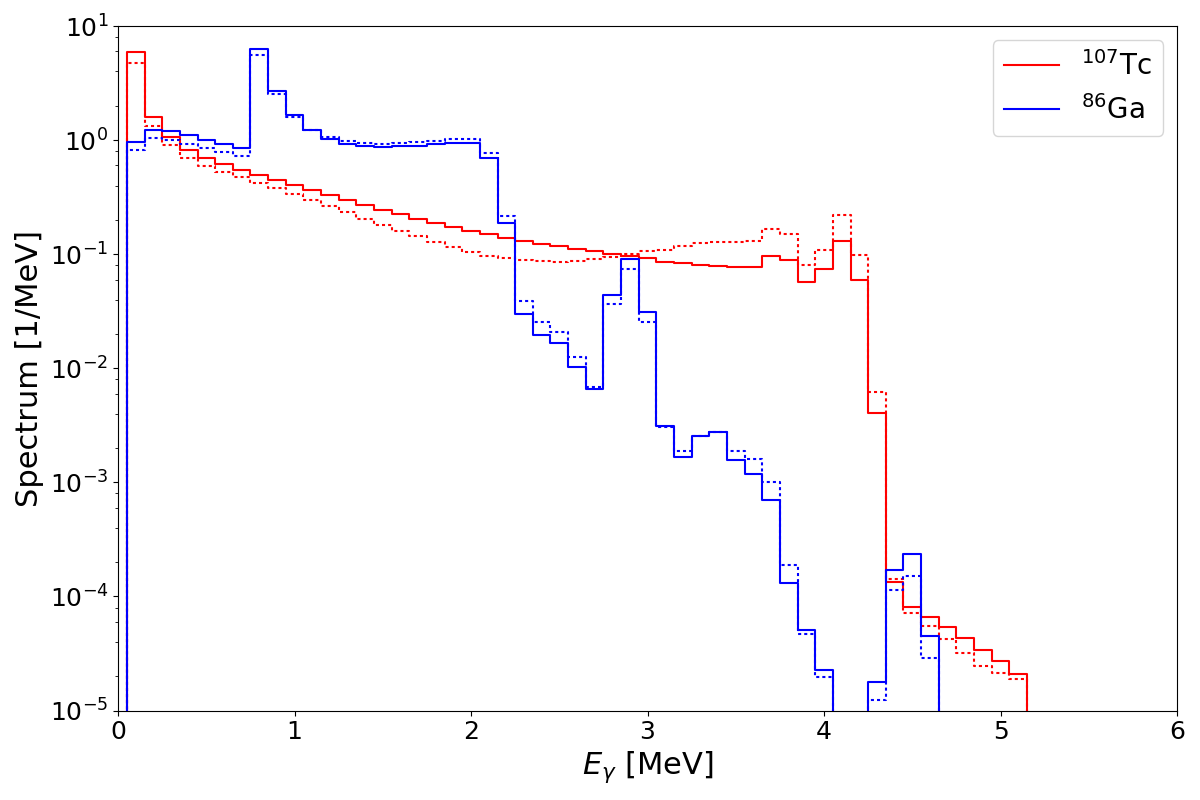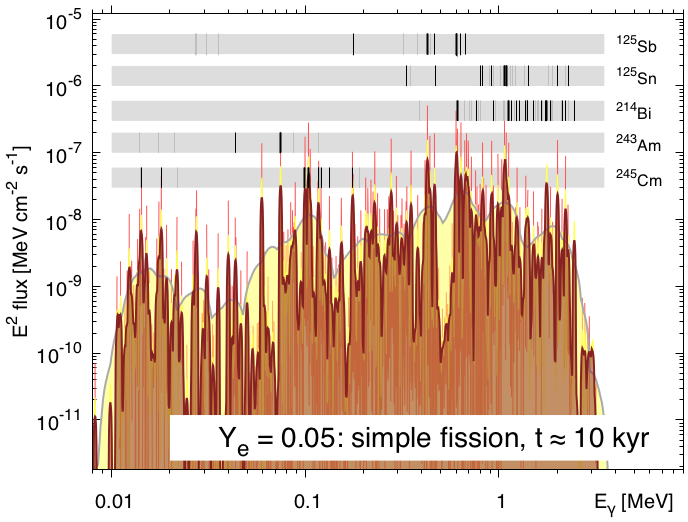Our QRPA+HF model is also capable of producing predictions of particle spectra coupled with evaluations (left)

Benchmarking these quantities provides a more sensitive test of the model beyond integral quantities such as $P_n$

Both theory & data are influential in predicting observational signatures e.g. $\gamma$-rays from remnants (right)

Measurements focused on decays can provide a new handle on potential 'smoking gun' $r$-process nuclei

$^{213,214}$Bi($Z=83$) are strong $\gamma$-emitters that have short half-lives but can be generated by longer-lived species

Korobkin et al. 889 2 (2020) • Miller et al. PRD 100 023008 (2019) • Wu et al. PRC 101 042801(R) (2020) • Mumpower et al. in prep. (2021)

## On even longer timescales...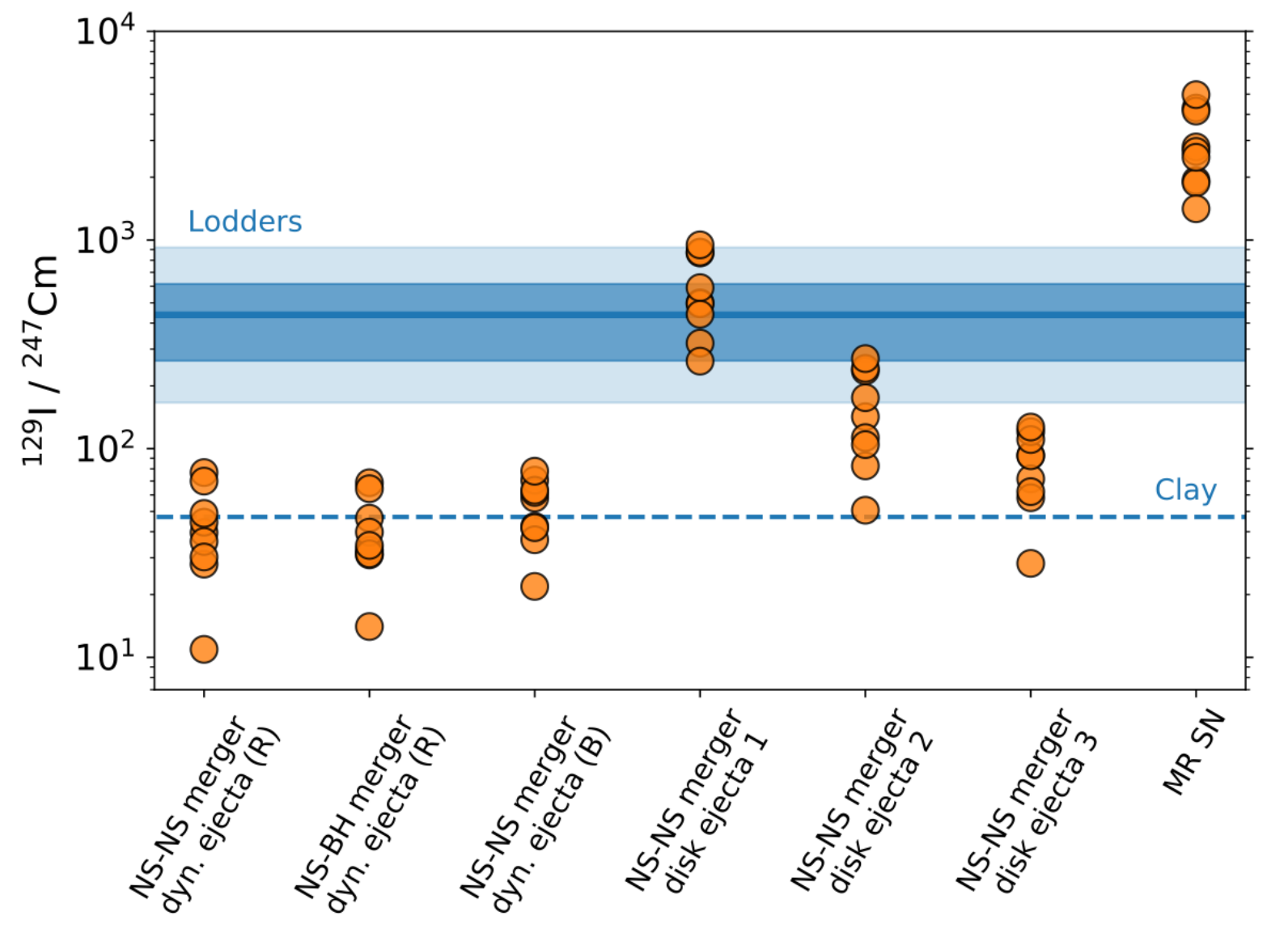The $^{129}$I to $^{247}$Cm ratio offers a unique insights into the $r$-process

These radionuclides have nearly identical half-lives (15 Myrs), but are widely separated in mass

See Benoit's talk on Friday!

Côtè et al. Science 371 945 (2021)

## Special thanks to

My collaborators!

## Summary

We need accurate nuclear data and reliable nuclear physics models to understand $r$-process nucleosynthesis

Providing novel insights into radionuclides of the $r$-process and their associated signatures in various epochs